Select Page

### Eventually into the Pyramid of the SunIn this section of our exploration of the Primary Frequency of the Constant 27.5, we will explore its intersection and interaction with basic geometry, the hyperdimensional geometry of the sefirot, how it radiates through the aether, and its relationship to Spherical Time and the Endless. We will also witness how its interactions with the concealed 112 Triplets in the Torah, along with the fields of Pi (π) and Phi (φ) gave rise to a very special and lasting blueprint with no input from Man whatsoever.

Nikola Tesla was obsessed with the number 3 and subsequently with 9 and 18.  He was also obsessed with frequencies. So were the ancient kabbalists.  The number 27.5 is a very special number, unique in how it interacts with the number 9. At its core it is a frequency that ties together all the other numbers.

As a basic mathematical principle or rule we can divide any number by any other number, except zero, which exists only as a placeholder.  Decimal places within numbers are just multiples or divisions of 10x thus we can basically ignore them.  So, considering the set of all whole numbers individually as our numerators, we can divide any of them by another whole number (the divisor).  For arguments sake, let us extend that to one decimal place, starting with 1, 1.1, 1.2…, even though we know that is really the same as 10, 11, 12.  What we find is an endless stream of results for 1/1. 1/1.1, 1/1.2…. 2/1, 2/1.1, 2/1.2….3/1, 3/1.1, 3/1.2….

Some of those fractions result in whole numbers like 4/2 = 2, or in exact terminating numbers such as 1/1.6 = .625. Some of those fractional results are irrational numbers, an endless string of seemingly random digits. Others result in repeated patterns of single numbers, like .11111…. or .444444…. Some have triple repeat patterns like 11/2.7 = 4.074074…. For this exercise, let us ignore the portion before the repeating begins because that is just a function of the size of the number. At a certain point, unless the result is irrational, all the numbers will settle into a repeat pattern. Many of the numbers will result in a 2-digit repeating pattern, such as .242424 or .424242 or .151515. Yet only a tiny set will result in 2-digit repeats that sum to 9, as in .090909…, .181818…, .272727…, .363636…, .454545…, .545454…, .636363…, .727272…, .818181… and .909090….

Before we begin analyzing this, we need to point out that the sum of .090, .181, .272, .363, .454, .545, .636, .727, .818, and .909 equals 4.995, as in the 4995-sum of the 27 letters of the Alef-bet.  If the decimal place is extended indefinitely as happens naturally, the result is 4.9999… = 5, the number at the core of the Torah (58) or 5 Books of Moses, and of Phi (φ), which is based on the square root of 5. As Tesla would ask, what generates this, what frequency in the universe generates these strings of 9 in the results of pure numbers?

As it turns out, there are only a few such numbers that can be used as divisors, the active part of the equation, to generate such results. They are 1.1, 2.2, 5.5. 11, 22, 27.5 and any multiple of 27.5 in the order of F(x) = 27.5(n + 2). At first glance that gives us exactly 8 frequencies through 110, and of them the number 22 stands out as reflective of the 22 Hebrew letters and the simple equation (27.55.5) = 22.   Yet on further reflection, we realize that F(x) = 27.5(n + 2) yields 55, 110, 137.5, 220, 275…, meaning that 1.1, 2.2, 5.5. 11, 22 are just derivative of 55, 110, and 220. Therefore, it is only by dividing a number by 27.5 or certain of its even number derivatives that we can generate strings of numbers with repeating sets of 9’s.

How interesting that the measure of the sacred cubit (27.5”) is a number that is mathematically unique amongst all other numbers, and that it is integrally connected to the number 9. Which makes it integral to the design and make of the Alef-bet, the Torah, and to Phi(φ).

It also makes the 27.5” sacred cubit unique amongst all other measurements that could possibly have been chosen. When the blueprints for the Mishkan, or Tabernacle of 42 Elements were given they came directly from the Creator.

Not every number that we divide by 27.5 will yield these special results based on 9.  The exception are the numbers that are derivative of 27.5 such as 1.1, 2.2, 5.5. 11, 22, 27.5, and any multiple of them. These will naturally wind up being whole or terminal numbers equal to the multiple from which they derive.

As it turns out, each of these special derivative numbers are exactly 11 units apart, just like the 11 sefirot or dimensions in the Tree-of-Life schematic of the universe, 11 covering 10. And the 11 sheets of the tent that covered the 10 tapestries of the Mishkan above its 10-cubit high beams. Every grouping of the 10 results between them ends in a complete set of repeating .363636…, .727272…, .090909…, .454545…, .818181…, .181818…, .545454…, .909090…, .272727…, and .636363… in that exact order, forever.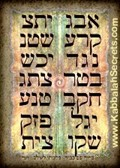A corollary is that any number that ends in one of these 10 repetitions is cyclically divisible by the frequency 27.5.  For example, the energetically masculine over the feminine portion from the Name YHVH (יהוה): YV (יו)/HH (הה) = .2909090… = 8/27.5, which is also the value (290) of the 27th and 28th letters—the final 2 letters (רץ)—of the Torah’s first verse. These are also the middle 2 letters (רצ) of the 42-Letter Name Matrix, and moreover the only letter pair that they have in common.

Their ordinal positions in the Torah add up to (27 + 28) = 55, an odd Pronic Number that equals 2 cubits (2 x 27.5), while their corresponding recurrences are (83 + 82), which works out to (27 + 83) + (28 + 82) = (110 + 110) = (8 x 27.5). We can thus see how the Creator wove His Name YHVH (יהוה) along with the Primal Frequency 27.5 into the Torah’s first verse and the 42-Letter Name, which respectively make up the 11 and the 14 Essential Triplets.

The 10 cyclical remainders are self-organized into 5 concentric pairs of 99: (.36 + .63), (.72 + .27), (.09 + .90), and in the center (.81 + .18), like the sum of the yields of the 2nd and 3rd normal magic squares (34 + 65) = 99.  The hidden pattern in these 10 endlessly repeating numbers that are responding to the frequency 27.5 is: (+36, -63, +36, +36, -63, +36, +36, -63, +36) or simply (+36, +36, –63).

The universe ordered these numbers this way, not our spreadsheets, and certainly not Tesla, and yet… When we apply the revelatory cipher of taking their square roots, this repetitive pattern shows some very interesting results for us and for Telsa himself.  The results are the same whether we use the square roots of 36 or .36, so for simplicity, let us use 36, 72, 90, 45, 81, 18, 54, 90, 27 and 63.  The results in order: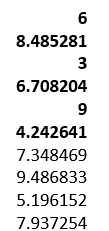The 3 6 9 results are highly recognizable from Tesla’s quote, “If you only knew the magnificence of the 3, 6 and 9, then you would have a key to the universe,” yet the 3 numbers they are interlaced with are highly significant to us and to the ancient Kabbalists.

While the 6th number on the list, 4.242641, the square root of 18, represents Moshiach Ben David and the Cosmic Consciousness (424), the YHVH (26), etc., the 2nd number on the list, 8.485281, is just that 6th number doubled.

The 4th number of the repetitive set of 10 is 6.708203… and 6708 is the exact gematria value of the 42-letter Sword of Moses found in Exodus 15:11 with the chapter/verse coordinates 15 and 11 being indicative of the 15 Triplets and the 11 Triplets on one of the two axes of the Alef (א) of Creation, as previously covered. The other axis being the 14 Triplets of the 42-Letter Name and the 72 Triplets of the splitting of the Sea, or 26 Triplets and 86 Triplets. The number 67 is also the numerical value of Binah; 708 of the Upper 42 Letters of the Name (יהוה) concealed with those 15 Triplets of the Shema; and the 203 is the value of the first of those 11 Triplets, the first Triplet in the Torah (ברא).

## מִי-כָמֹכָה בָּאֵלִם יְהוָה,  מִי כָּמֹכָה נֶאְדָּר בַּקֹּדֶשׁ;  נוֹרָא תְהִלֹּת, עֹשֵׂה פֶלֶא

Of those derivative frequencies (1.1, 2.2, 5.5. 11, 22, 27.5) of 27.5 that gave us the series of the resultant 9’s, the number 27.5 is the 6th one, as in the 6 handsbreadths that we have been told by definition equal 1 cubit.

The cycle of resultant 9’s is in sets of 10, falling in between every 11th derivative number, which matches our 10 sefirot with a separate 11th sefira dimensional model of the Tree-of-life, and also thevibrating string theory model of the universe.  While this repetitive pattern is endless, there is one distinct set of them that we can highlight.  The first numbers that result in the exact repetitions in ascending order from .0909 to .1818 through to .9090 form a special and unique set. They correspond to the numbers 25, 50, 75, 100, 125, 150, 175, 200, 225, and 250, incremental by 25.  And the sum of the 10 repetitive 9 multiples (25/27.5, 50/27.5…250/27.5) work out to exactly 50, while the sum of the series (25, 50…250) is 1375, recognizable as 50 cubits of 27.5”, as in the 50 Gates of Binah, the 50 cubit height of the Gates of the Holy Temple, the 50 cubit height of Noach’s Ark, the 1375-value of the last 3 lines/levels of the 42-Letter Name Matrix, and the Phi (φ) angle (137.5o).

### The 9 x 9 Magic Square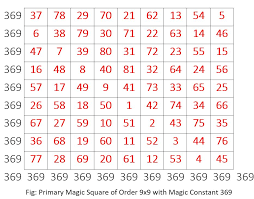The 3 6 9 results are highly recognizable from Tesla’s quote, “If you only knew the magnificence of the 3, 6 and 9, then you would have a key to the universe,” yet the 3 numbers they are interlaced with are highly significant to us and to the ancient Kabbalists.

The numbers 369 are also found in the 9 x 9 normal Magic Square whose rows, columns, and diagonals equal 369. This is 258 more than the 6 x 6 Magic Square of 111, as in the (42 + 216) days of the Cosmic Wheel that lead up to the Day of Creation, and as in the diagonal (258) of the 3 x 3 normal magic square.

Together, the 3 x 3, 6 x 6, and 9 x 9 normal Magic Squares equal (15 + 111 + 369) = 495. This is the sum of the 10 remainders 36, 72, 90, 45, 81, 18, 54, 90, 27 and 63 that equal 495, just like the sum of the 18 values of the Hebrew letters from Alef (א) to Zadi (צ) which are found in the .333, .666, .999 split of those letters. It is also the 495o turned in the Spherical Time paradigm every 10 seconds because of Phi (φ), which works out to (18 x 27.5), or (3 + 6 + 9) x 27.5.  Moreover, the sum of the digits in 495 = 18, giving it a P/S cipher of 180/18 = 10.

The Alef-bet is structured pyramidal in 3 tiers of 9 letters whose values increase geometrically from 45 to 450 to 4500, according to the value of the first letter in each tier (איק) or 1, 10, 100 respectively, as reflected in the 6 x 6 Magic Square of 111.

This structure of 45 based on the sum of the integers from 19 is also reflected in the 10 quotient 27.5 remainders (.363636…, .727272…, .090909…, .454545…, .818181…, .181818…, .545454…, .909090…, .272727…, and .636363…) whereby each subsequent digital layer is also the sum of 19 and thus 4.5, .45, .045

The ordinal value of the initials (איק) for each tier is 30, and the ordinal value of the final letter Triplet (טצץ) is 54, for an average of (30 + 54)/2 = 42, which makes intuitive sense since the average of the total ordinal values is 378 and 378/9 = 42 and thus all the Triplets pairs average to 42, with the middle or 5th Triplet (הנך) being 42.

The 6th Triplet (וסם) naturally has a value of (6 x 111) = 666, and an ordinal value of 45, giving it a complete value of 611, as in Torah (תורה).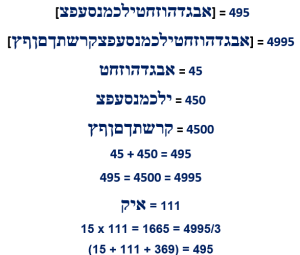While the sum of the 3 x 3, 6 x 6, and 9 x 9 positions in the 3 normal magic squares equals 126, and average (126/3) = 42, as in the faces’ and planes’ values (126) of the Essential normal Magic Cube of 42, their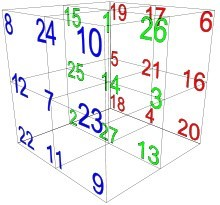total value is 4032, giving them an average positional value of (4032/126) = 32, as in the 32 Paths of Wisdom built into the Tree-of-life and the 322 word values in the Torah.

The value 45 inherently built into the 3 tiers of the Alef-bet is the standard numerical value for Man, or Adam (אדם), which is an acronym for Adam-David-Moshiach, the path that traces the Spherical Time Clock from the birth of consciousness on our plane to Cosmic Consciousness. The complete sofit value for Man or Adam of this potent acronym (אדם) is (605 + 28) = 633, as in digit #633 in Pi (π), where we first encounter the numeric string …5778….

The number 369 comes up again in the result of multiplying the 304,805 letters by the 79975 words by the 5845 verses in the Torah to get 142482278369375. Normally, a three digit number appearing in such a large number would be somewhat coincidental and it may be here has well, except that the first four digits (1424…) is the value for the “Holy of Holies”; and the next set of four (…4248…) is the sum of the 22 Names (142482278369375) of the Alef-bet; followed by 278, the value of Ohr HaGanuz, the light of Moshiach Consciousness; with the last four digits (…9375) being the difference between the sum of those letters, words, and  verses and 400,000 or (400,000 390,625) or (15 x 625).

### The Magic in the 3 – 9 Normal Magic Squares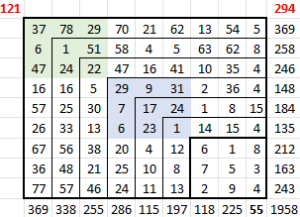If we take the initial 3 x 3 or 32 normal magic number as a starting grid and expand it one layer at a time to 42, 5292 by adding the corresponding layer from those normal magic squares we get a new 9 x 9 grid that is no longer a magic square but is composed of all of them and consequently has some inherent magical properties.

The most notable one is that the sum of the first column totals 55 and only 55. There is no inherent reason for it being 55 or (2 x 27.5) except by cosmic design, and no reason whatsoever that the total should be so low, even the next column over is 170 more, or 225, which is interestingly enough 152, like the yielded constant of the first 3 x 3 magic square, and the sum of the yielded constants of the 32, 42, 52, and 62 normal magic squares.

The sum of the first and last columns (55 + 369) = 424, as in Moshiach Ben David, the Cosmic Consciousness, while the rest of the columns equal 1534, like the first two normal magic square constants, 15, and 34.

Then we have the diagonals of 121 or 112 and 294, as in the 294 times the YHVH (יהוה) appears with prefixes in the Torah.

Then there is the sum of the 81 square roots equal total to 358, as in Moshiach, and the sum of the 3, 4, 5, 6, 7, 8, and 9 squares that equal 42.

When we add those 81 positions to the sum of the (3292) normal magic squares we get (15 + 34 + 65 + 111 + 175 + 260 + 369) = 1110 or 10 x Alef (111) and 10 times the middle or 52 normal magic square (111). Moreover, the difference between the total value of the 7 normal magic squares and the combined one is (77981958) = 5840, very similar to the 5845 verses in the Torah.

When we form a new 3 x 3 square in the center of the 9 x 9 one, we get a square centered on the number 17, whose total value is 147, as in Jacob’s 147 years and the 147 value of the 7 Ehyeh (אהיה) in the Torah and with its diagonals (101) its full total value is 248, as in Abraham and the 248,000φ elements in the Torah. What makes this more interesting is that the 10 x 10 normal magic square has a yielded constant of 505 as in the numerical value of Abraham’s wife, Sarah, and when we add 505 to the total for the first 7 normal magic squares, we get 1534, like the first two of them, 15, and 34, and like the 7 central columns in the 39 combined square.

The next 3 x 3 square in the progression is centered on the number 1 and its diagonals total 137, while its total value is 432, as in the radius of the Sun, etc. The sum of the diagonals in all 3 sets of 3 x 3 squares that represent .333 of the total 9 x 9 square is 267, as in the hypotenuse of the Tower of Truth, which we will get to shortly.

The 11 x 11 normal magic square has a value of 671, which as the Arizal explained is the value of the Name Adonai (אדני) when filled in (אלף־דלת־נון־יוד) and is like the 671 million mph speed of light.

### The Mysterious Sequence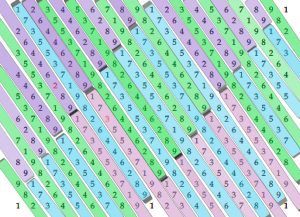As discussed in previous articles, there is a mysterious palindromic 18-digit sequence (173553719826446289) built into the fabric of our universe. It is in our base 10-digit stream; the Fibonacci Numbers using mod 9 for every 4th number; the Tower of Truth by taking the 21st cube (213) as a percentage of all 21 cubes from 13213; the continual numeric torus as illustrated above in an unwrapped section of it, and now we see that it is tuned to the frequency of 27.5.

The diagonals in this toroidal wrap of the repeated 18 digits 19 in one direction and 91 in the other give us the amazing and mysterious repetitive sequence 173553719826446289.

The diagonals in the opposite direction (181818, 272727, 363636, 454545, 545454, 636363, 727272, 818181, 999999) give us the remainders we encounter when we divide numbers by 27.5.  Please note that while .090909 and .909090 cannot be present in this dynamic because there is no digit “0” in the schematic, together they are jointly represented here by (.090909 + .909090) = .999999.

While the repeating sequence 173553719826446289 derived from mod 9 of the Fibonacci Numbers is based on the number 9, we see that when the two palindromic sequences are added using zero (0) as a placeholder, as in (0173553710 + 9826446289), they total 9999999999. In other words, each complementary digit pair (1 + 8, 7 + 2, etc.) in the sequence sums to 9.

Then when we split the 18-digit mysterious sequence (173553719826446289) into 9 sequential pairs (1+7, 3+5, 5+3, 7+1, 9+8, 2+6, 4+4, 6+2, 8+9) and then reduce them to small gematria, we get (8+8+8+8+8+8+8+8+8) = 72.  Keep in mind that to get these 18 repetitive numbers, we took every 4th Fibonacci Number, meaning the sequence repeats every (4 x 18) = 72 Fibonacci Numbers. That is the beauty of a coherent design Created in a mind that can see infinite symmetry and know how all things are connected.

When we divide the two directions in the torus by each other (123456789/987654321) we get .124999999, which is the fraction 1/8th and is possibly related to the sequence at the 756th digit in Pi (π), as in the 756 value of 27.52: …721134999999837297804995…, which begins with 72, includes 4999999 and 729 or 93 and ends with 4995, the sum of the 9 x 3 letters in the Alef-bet.

Its inverse is (987654321/123456789) = 8.000000729 with 729 equal to 93, the value of the second level of the 42-Letter Name Matrix.  Another related equation between the two directional digit streams in the torus that ties in the 8 and 9 elements is (123456789 x 8 + 9) = 987654321.

Thus, we see the frequency of 27.5 interacting with the simple digits 19 yet again in the simplest and most wondrous natural ways.  And while the digits in 19 and 91 sum to 90, so do the digits in 173553719826446289 and so do the repetitive digits in the diagonal sequence and in the 10 remainders.

### 213/ (Σ(13 – 213) = .173553719826446289

The first apparent Torah connection to 173553719826446289 is found in the total gematria value of all the words in the Torah, 21009826, which itself relates to the Tower of 212 and to sum of the 3 consecutive normal Magic Squares of (4 x 4), (5 x 5), and (6 x 6), that equal (34 + 65 + 111) = 210 and whose digits equal 21, which is also the height in cubits of the Tower of Truth whose volume is 21 x 210 cubits. Then there is the …9826… in 21009826 and at the center of the mysterious sequence 173553719826446289, but just as eye-catching is that (21009826 17355371) = 17.3% of 21009826, as in the gematria katan of the 42-Letter Name, 173.

While the 20 stages or stacked sequential cubes (203 + 193 + 183… + 13) of the Tower of Truth have a combined height of 210 cubits, which represents the sum of the first 20 integers, the cumulative sum of the first 20 cumulative Prime Numbers is 4200 or (20 x 210).

As astonishing as this all seems, it is even more mind-boggling that the square root of the 58 words, letters, and verses in the Torah, or 625—the value of the highest dimension, H’Keter—when divided by the square cubit (27.52) equals 1 – .173553719826446289).The secret code of Creation directly relates to the mysterious palindromic 18-digit sequence (173553719826446289) built into our base 10-digit stream, the Fibonacci Numbers, the Tower of Truth, and who knows where else it will turn up.  And they all relate through the Torah (58), the Primal Frequency of the aether (27.5 Hz), and through Phi (φ).

If we add the 183 cube or 5832 verses in the Torah to the 378 or 33 Essential Magic Cube of Creation, we get (5832 + 378) = 6210, and 173553719826446289/Phi(φ) = 10726210, which leads us to the Event Horizon 5778 as the 107th Triangular Number and to Phi (φ)18 = 5778.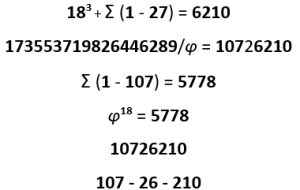Extending the process, we get 173553719826446289/5778.28 = 3.00355330352. In this result we see the year 5778.28 tied to 3 and to the 3003 total of the 11 Triplets of Bereshit; and to the value of the Hebrew word for year, Shanah 355 that is also found in 173553719826446289 right after the 173 katan value of the 42-Letter Name that begins the sequence, along with the 3553 times that the letter Alef (א) is used as a final letter in the Torah.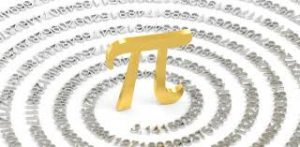Moreover, at the 173rd digit in Pi (π) is the numeric string …2110555… which is the number of days in 5778.28 sidereal years, while the digit sequence …300355.. of the resultant 3.00355330352 is found in Pi (π) at digit #1358, as in the numerical value of the Baruch Shem that should be recited silently after each of the 5 Essential Elements of Creation to help engage our minds to them and to Binah (1000), the higher Consciousness level, coupled with 358, Moshiach Consciousness, the Cosmic Consciousness.

The value 1358 is also the sum of the fully spelled-out Names YHVH (יהוה), Ehyeh (אהיה), and Adonai (אדני), (232 + 455 + 671) fusing all three levels.

The first occurrence of 5778 within PI (π) occurs are digit #633 and its fuller sequence is …4275778…, connecting 42 with the event horizon in 5778 and with the cubit (27.5) and Primal Frequency (27.5 Hz).

An odd alignment with that is found in the standard gematria of the entire Torah, 21009826 divided into cycles of 27.5 Hz, which works out to (764,000 x 27.5) less 174 or (6.33 x 27.5). Compensating for that 6.33, the total would be 21010000. These 27.5 cycles or intervals of 27.5 are exactly 100,000 less than the 864,000 mi diameter of the Sun.

The gematria of “The One (האחד)” is 18 and we see how the 18-digit mystery sequence that adds up to 90 is keyed into the frequency of the universe, Pi (π), the Torah, and Spherical Time all at once through the equations: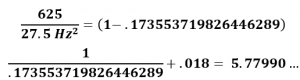While the 3 strings …5778… found in Pi (π) are connected directly to 18, the subsequent year 5779 is found represented 3 times as well through the string …2019… as in 2019 CE within those same first 1000-digits of Pi (π). Meanwhile, the log of 601,730, the precise second census number given in the Torah for the Israelite men who completed the 42 journeys to reach the Promised Land, is 5.779.

The angle 90o gives shape to physicality. So, the complementary angle to 27.5o is (90o27.5o) = 62.5o indicating that physicality is determined by the Eminences of the Creator, H’Keter (625), and its emanating frequency (27.5) in the aether that drives all the other fields.

The ratios between them, 625/27.52 = (1 – .173553719826446289) and 625/27.5 = 22.727, and (625/27.5 + .173) = 22.9, define further that the relationship and include the Source field of the 42-Letter Name (173).  It is the equivalent to the space/time ratio that is also the ratio of the cubit to the foot 27.5/12 = 2.29.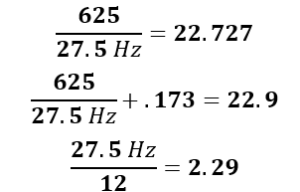Moreover, the square of this relationship is 516.516, as in the  516 times that Moses had to pray to bring about the final redemption. This apparently relates to the 42-Letter Name that Rav Brandwein of blessed memory advised is linked to that same final redemption once we understand it.It might also relate to the diameter of the Sun’s orbit around the Milky Way Galaxy of 51,600 Light years.

### Sourced to the OneWithin the digital torus, we see this sequence repeated endlessly like an interlocking puzzle piece or tessellated tiling: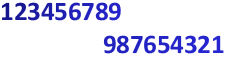What we need to understand about the mysterious 173553719826446289 sequence that is derived from this tiling of 12345678987654321 is that it is ultimately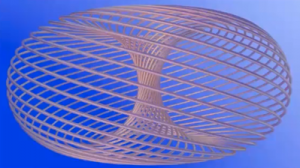derived from One (1), just like Phi(φ), since the square root of 12345678987654321 = 111,111,111. In metaphysical and hyperdimensional terms squaring is eminence, radiance from a source state. A Number reveals its state and position, and in analyzing the essence of a number we often examine its square root or cube root or even higher root power, like seeing that the square root of the Torah is 625 and that its 8th root of the combined Torah elements or networked matrices is simply 5.  This tells us much more about its position and hierarchy in hyperspace vis-a-vis the Creator and Ultimate Source. The only reason to do this is Understanding which brings us in closer alignment with the Source and the Creator. When we are able to resonate with Understanding, we no longer have a need for physicality.

One is the Source.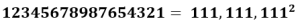###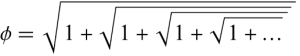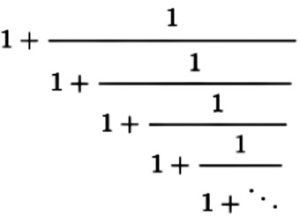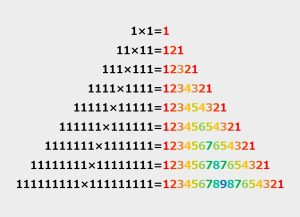Why do we keep talking about matrices and vectors as they apply to the 5 Essential Elements of Creation, the 4 sets of Triplets Matrices and the 26 vectors within Magical Essential Cube of Creation? First, the concealed sets of prearranged letters and the ciphered values that form these Matrices are mathematical or numeric coordinate spatial grids, and the vectors radiating spherically from the center of the Essential Cube of Creation are rays drawn through two points that are also coordinates in space. To keep it as simple as possible, matrices can be applied to vectors to scale and/or rotate them, giving them all new coordinates and thus new direction and length.

In the physical world this has myriad applications such as in algorithms; network analysis, including neural networks, machine learning and AI; linear equations, especially ones with numerous circuity like electronics; dynamic systems; digital photographic recognition, processing, or analytic software; and image projection across dimensions like projecting a cube or sphere into a 2-d plane.

And in spirituality and hyperdimensional mathematics distance in space is determined by similarity of form. The more alike we are to the attributes of the Creator, the closer we are to Him. So, in the metaphysical world, being able to shift within the Cosmic neural network of universal consciousness is akin to limitless potentiality.

So yes, those 5 Essential Elements are of critical importance to the structure and processing of the Cosmos, including our consciousness.

### The Primal Frequency and 3

As it happens the 210 cubits in the Tower of Truth of 27.5” is 5775” in height or 3” shy of the Event Horizon radius of 5778.  In a very similar way to which we reached 4/π, the cosmic harmonic (1.273), using the set of all odd numbers, we can reach 3 using only 27.5.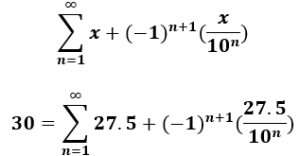This fancy looking formula is a recurrent progression such that value 27.5 increases and decreases by an ever-smaller amount (27.5 + 2.75 – .275 + .0275 – .00275 + .000275…) until it eventually reaches 30.00000000000000000… In other words, oscillating reflections of itself.

This may be why the recurrent progression formula fits the design of the sacred cubit and the Tower of Truth so perfectly.

Moreover, 30 is the numerical value of the letter Lamed (ל), and its complete value is (12 + 30) = 42.  This is like the 42 repetitions of the name Yehuda (יהודה) of numerical value 30 in the Torah. The Name Yehuda (יהודה) is special because it contains the YHVH (יהוה).  Like the value 21 associated with Binah through the Name Ehyeh (אהיה), the letter Lamed (ל) is the one letter associated with the level of Binah, as it is the only letter designed to reach upwards beyond the physical plane. There are 5 letters that reach below the plane (קךןףץ) and their collective value is 3000.

By shifting the decimal place in the equations, 30 becomes 3 and not only is the Primal Frequency aligned with the number 3, but the height of the Tower of Truth, 5775” is compensated for and adjusted to 5778”, the Event Horizon of Spherical Time.

### The Tower of Truth and the Tree of Life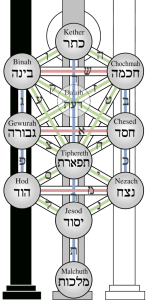That Tower of Truth is 210 cubits of 27.5” each tall, which matches the 3 final letters (ההר) of the top 3 sefirot in the Tree-of-Life schematic—Keter (כתר), Chochma (חכמה), and Binah (בינה)—that add up to 210 and spell out “The Mountain(ההר).”

While the first 3 final letters (רהה) add up to 210, the last 3 final letters (דדת) of the 11 sefirot of the Tree of Life add up to 408, as in the (600,000408) component letters in the Torah. Together those 6 bookend final letters add up to (210 + 408) = 618, or 1/Phi (φ).

The Tower of Truth is more than a metaphor. It is a link between worlds, a portal between dimensions. Its dimensions are the key. Its connection to the upper realms of the Tree-of-Life are not casual; they are designed. Every aspect of the Tower of Truth was meticulously designed. The connection to “H’Har The Mountain (ההר)” is not coincidental.

Is it also by design that the front letters (בינ-חכמ-כת) in those 3 highest sefirot equal 550 or exactly 20 cubits (20 x 27.5”), that in turn matches the initials of all 11 sefirot (כחבדחגתנהימ) which sum to the same 550 or (20 x 27.5).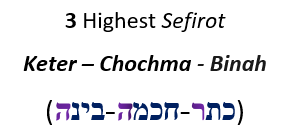Meanwhile, of the 42 letters in the 11 sefirot, the first 5 initials (כחבדח) of the 11 sefirot sum to 42, while the last 3 (הימ) sum to 55 or (2 x 27.5), which is 1/10th of the full 11, and they also make up the final 3 letters in the Name Elohim (אלהים).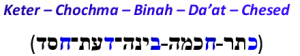This is appropriate considering that “The Path of the Tree-of-Life (את־דרך־עץ־החיים)” has its 4 final (תךץם) letters equal to 550 as well, while “The Path (את־דרך)” has a gematria of 625, as in the value of H’Keter (הכתר).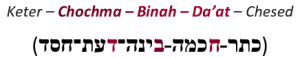The initials of the 5 uppermost levels of the Tree-of-life dimensions equal 42. The level of Keter is unattainable to us as it is the level of pure Source. The next 3 levels—Chochma, Binah and Da’at (Wisdom, Understanding and Knowledge)—form the upper worlds beyond the limitations of physicality. This is why their initials total 14, as in the central position of the Essential Magic Cube of Creation of 42. This is the position from which the 26 vectors emanate. Chesed is the first of the 6 bundled dimensions of Zeir Anpin and is equivalent to what we call Heaven.

The 6 dimensions of Zeir Anpin are typically represented by its central sefira (dimension), Tiferet (Beauty), which gives the 4 serifot of the central axis or trunk of the Tree of Life (Keter-Da’at-Tiferet-Yesod) the numerical value of 424 for their 4 initials, as in Moshiach Ben David, the Cosmic Consciousness, and as in the 4 letters of our DNA, but more on that shortly. The initials of Moshiach Ben are MB (מב) or numerical value 42 and David (דוד) has the value 14 the central position of the Magic Essential Cube of 42.

Since the sum of the 11 initials (כחבדחגתנהימ) equals 550 or (20 x 27.5), and the Trunk of the Tree equals 424, the remaining 7 branches equals (550424) = 126 or (3 x 42), as in the value of the faces and planes of the Essential Magic Cube of Creation. Moreover, when separating out the 11th dimension of Malchut, whose initial (מ) has a value of 40, the 6 branches equal 86, as in the Name Elohim (אלהים).

Malchut (Kingdom) is the final or 11th dimension and consists only of the joint projection from the 6 dimensions of Zeir Anpin. It is an illusion; it is the illusion we call physicality. Its numerical value is 496, the sum of the cumulative verses in the first 6 Days is (15 + 21 + 55 + 99 + 86 + 220) = 496. It is the perfect 3-d illusion, and 496 is the 3rd Perfect Number. The first Perfect Number is 6, while the second Perfect Number is 28, as in the paired values that surround the central 14, and as in the pair of initials of Keter and Chesed that surround the upper 3 dimensions of 14. It is no coincidence Pi (π) begins 3.14….

The 42-letters are likewise split into the 4 upper dimensions of 14 letters and the lower 7 dimensions of 28 letters.

Since the last 3 initials (הימ) of the 11 sefirot sum to 55 as in the Name Elohim (אלהים), and since there are 42 letters in the 11 sefirot we should note that there are 31 letters in the backs of the 11 Names of the sefirot, as in the first 2 letters (אל) in the Name Elohim (אלהים).

Moreover, the total 550 for the 11 initials less the final 3 is (55055) = 495 or (202) x 27.5, which is also (.9 x 550) as in the 3 x 3, 6 x 6, and 9 x 9 normal Magic Squares that equal (15 + 111 + 369) = 495; and as in the sum of the 10 remainders 36, 72, 90, 45, 81, 18, 54, 09, 27 and 63 from dividing by (27.5) that equal 495; as in the sum of the 18 values of the Hebrew letters from Alef (א) to Zadi (צ); and as in the 495o turned in the Spherical Time paradigm every 10 seconds. Everything is connected through specific networks and pathways. Everything has a purpose.

### Planck’s Constant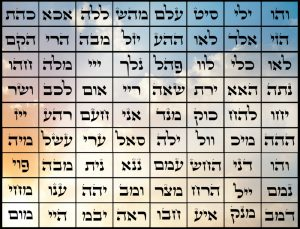Another connection to the value 210 beyond the 210 cubits height Tower of Truth and the 21009826 total value of the Torah is found in the diameter of the Planck’s constant h/π or 21.0914362859 x 10-35 that consists numerically of 210, and 9143, which is the sum of the 216 letters in the 72 Names (Essential Triplets), and of 628 or 2π.

The Spherical Time equation for the circumference of the Spherical Time Bubble of radius 5778 years, is also based on the formula for the surface area (4πr2) of the two spheres of Time, Physical and Spiritual. Given that their two radii 2018/2 + 3760/2 add up to 2889, the midpoint in King David’s life, our original formula reduces to:

### 4π (2889)2/2889 = 36304.24470

Which then reduces to:

### 4π (2889) = 2π (5778) = 36304.24470

Planck’s constant h is the circumference of a sphere with a diameter of h/π and a radius of h/2π. Combining the two series of equations concerning the Planck length sphere and the Spherical Time bubble, we see that h/π or 21.0914362859 x 10-35 divided by 2889 equates to h/2889π = .0073006010… x 10-35 or 73.006010… x 10-31, aligning with the second highest sefira (dimension), Chochma of numerical value 73 that represents the value of Torah’s first verse.

Following the Spherical Time Equation for the surface area, and thus dividing Planck’s Constant (h) by 2889 gives us h/2889 = .022935514… x 10-35 or 2.2935514… x 10-33 which is similar to the harmonic space/time cubit relationship 27.5/12 = 2.291666 between the cubit and the inch. Obviously 2h/5778 = 2.2935514… x 10-33 as well.

Applying gematria to h/2889 = .022935514… x 10-35 we see that 355 is the value for the Hebrew word Shanah for year and 14 for David and thus the “year of David” which was 2889 HC, or 35 years after his birth and 35 years for his death.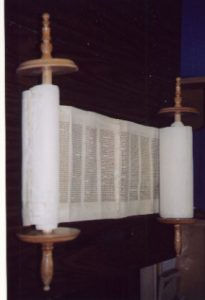These are not exact numbers, but due to the tiny size of the Planck constant, they are off by only .000000000000000000000000000000000018848 with 18 being the square of 4.2426 and 848 being (2 x 424.) Like Pi (π) and Phi(φ), Planck’s constant is not a particle; it is a relationship, another field designed to interact with the rest of the fields that define existence.

Moreover, the Primal frequency of the Planck’s Diameter is (27.5 Hz x 21.09143628 x 10-35) = 580.0145…, as in the Torah’s total gematria sofit of 5800.0542

The Planck scale is indeed tiny but no less important to the Creator than the cubit, foot, inch, mile, or AU.

### The Anomalistic Month

The harmonic time ratio of 27.5/12 is 2.29, yet on the 3-dimensional or physical scale it expands to (27.5/12)3, which is 12.0 to 1, which in turn represents the 2-dimensional ratio of the foot to the inch, 12 to 1. More precisely it is 12.035228 to 1. The value 120, the Age of Man and Moses, is both the sum of the first 8 Triangular Numbers, in other words the 8th Tetra Number, and 5! factorial.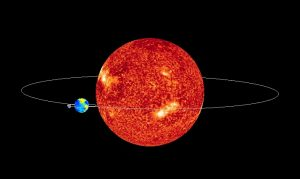As the Moon orbits the Earth it is not exactly a circular motion, but an oval, and each monthly orbit around the Earth, there is a point where the Moon is closest to the Earth. At that point it is moving faster than at any other time.  When we speak of months, there are different perspectives.  There is the sidereal month, which is how the Moon would be observed against the background of the stars, as opposed to our perspective from Earth. That sidereal month is approximately 27.32 of our days long, once again matching the Cosmic Harmonic (.273).

Since the Earth itself is independently moving at 66,600 mph around the Sun, the Moon must travel farther just to keep up with it, as it completes its orbit around the Earth.  Thus, what we observe as we watch the Moon go through its relative phases is that it exhibits a slightly longer month of 29.53059 Earth days, hence the lunar calendar of 29.5 x 12 = 354 days.

The anomalistic month is yet another variation.The lunar orbit does not occur at a steady rate, but rather the Moon speeds up as it approaches the Earth and slows down when it gets further away. The anomalistic month is the measure of the Moon’s quickest motion to the next occurrence of that quickest motion; in other words, whenever it is at perigee or closest to Earth. This average interval of successive perigees is 27.555 days, synching it with the Primal Frequency of the aether.

One could say that the anomalistic month, 27.555 days, reflects both one (1) and two (2) cubits (27.5 and 55 inches respectively), and with the full 27.55455 days, the time period further aligns with the 455 total of the 3 Aspects of higher Name Ehyeh (אהיה) which represent the 3 levels of Binah hyperspace, the World to Come.  The anomalistic year works out to 12.0085 months.

#### 12 cubits = 330 inches### Phi(φ), the Cubit and Primal Frequency, and 72

We have shown that the ratio of the cubit to the inch is 27.5 to 1, and the foot to inch is 12 to 1, and that the number 27.5 can be derived from the 4 Aspects of the YHVH (יהוה), and again through other either Divine or advanced knowledge derivations.  The cubit to foot ratio would be thus 27.5/12 or 2.291667. A cubit is supposed to be 6 handsbreadth and a rod is 6 cubits. Either way, 6 of those cubits-to-feet ratios would be thus 6 x (27.5/12) = (6 x 2.291667) = 13.75 ft and 6 ft is 72 inches.

Thus one (1) rod = 13.75 feet, and each rod is 1/10th the Phi (φ) angle (137.5o).

As previously explained Phi (φ) creates infinity out of finite space.  Yes, that is impossible in Euclidean geometry, but in a sense it is true.  It is very important to grasp this to comprehend why the universe and the most fundamental movements would be structured around Phi (φ). We already know Phi (φ) generates the most efficient use of space within our universe.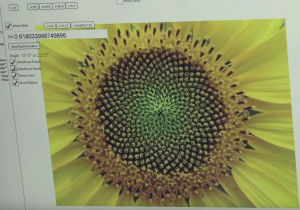As we spiral steadily and naturally outward utilizing the Phi (φ) angle (137.5o), elements, or points in space, will be deposited in the most efficient way possible. That can go on infinitely and so can adding points to a line, but what if instead of allowing the spiral to escape, we confined it to a simple 360o circle or to a specific radius, or with the toroidal shape that we see generated with the dielectric and magnetic fields.  We are not speaking about an ever-widening circle; we are speaking about a circle with a set radius, a set circumference. It could be a radius 1 or of 25, it does not matter. If the radius is limited, it is limited space. The same premise holds with the surface of a fixed radius torus.

When we use our plotting generator and advance incrementally by the Phi (φ) angle (137.5o), each time instead of recording where the next end point in the spiral will be, we record where the angle crosses the fixed circular plane, the circle.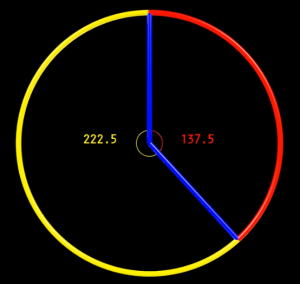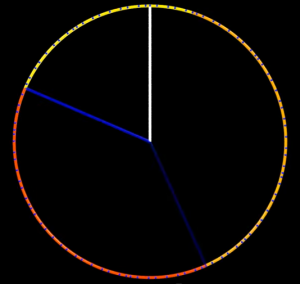Sure, we may have to draw finer and finer points, but no two points will ever overlap. The Phi (φ) angle (137.5o) will not intersect the circular plane at the same place twice. It will infinitely generate new points along the circle. No other angle will do that. It is unique.

This overlooked property of Phi (φ) has enormous implications and may be why the Creator chose it as a multiple of the Primal Frequency and thus of the sacred cubit. The Primal Frequency is 1/5th the Phi (φ) angle (137.5o), or (137.5o/5), which is the same as (2 x 13.75) = 27.5. It is also probably why He designed the Alef-bet to align with the Phi (φ) angle (137.5o).

The Phi (φ) angle (137.5o) splits the 360o circle into two proportionate parts. The larger one being 222.5o, their difference is (222.5o137.5o) = 85o.

We have just seen how those 6 bookended final letters of the 11 sefirot of the Tree-of-life add up to (210 + 408) = 618, or 1/Phi (φ). Their corresponding initials (כחב) and (הימ) on the other hand are 30 and 55 respectively, corresponding to the 22nd Triplet (ייי) and the 48th Triplet (מיה) of the 72 Triplets. They add up to (30 + 55) = 85. The initials (הימ) of Hod-Yesod-Malchut are a permutation of that 48th Triplet (מיה).

While the Primal Frequency (27.5) is 1/5th the Phi (φ) angle (137.5o), the value related to the 72 Triplets, 72o, is 1/5th of the full 360o. The angle 72o is 13o less than the difference of (222.5o137.5o) = 85o. When extended to the circle of 25,920 years in the Great Precession and to the circle of 25,920 parts of a minute in the Great Day, the ratio 72/25,920 is exactly 1o.

If like 72o of a circle (360o) we take the same 1/5th proportion of the circle of the Great Precession and Day, we get 25,920/5 = 5184, which is exactly 722.

### The Tower of Truth Begins to Take Shape

If we then take that 5184 result as a slope angle (51.84o) and extended it to the exact height of the Tower of Truth, 210 cubits high, the resultant base measure formed would be 165 cubits, which also equals 27.52/2or 378 feet, connecting on the one hand to (6 x 27.5) = 165, the small gematria0 value of the 42-Letter Name, and on the other hand to the 378 value of the 33 Essential Magic Cube of 42, that implies “His Name is One.” It makes metaphysical sense since 51.84 = (8 x 6.480…) or 8 times the square root of 42.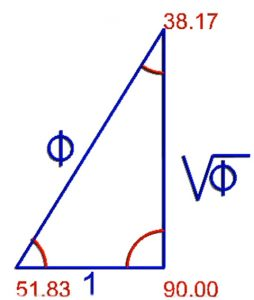Moreover, the difference in this right triangle to the top of the Tower of Truth between its height and base is (210165) = 55 or (2 x 27.5).

It all comes together, though, when we consider Phi (φ). To begin with, 72/27.5 = φ2.

Next, 72/φ = (72 27.5).

Then (72 27.5)/2 = 22.25, as in the Phi (φ) 222.5o angle, and by simple math 5(72 27.5) = 222.5.

Finally, 72/27.5 = (1 1/φ).

The relationship between the Singularity and Name of 42 and the YHVH (יהוה) is also Phi (φ) related in that 26φ = 42, and when the (222.5o137.5o) = 85o difference is split in half, it equals 2 equal parts of 42.5o, as in the value (425) of the 8th of the 72 Triplets (כהת), and the 425 verses in the Torah’s first 3 portions.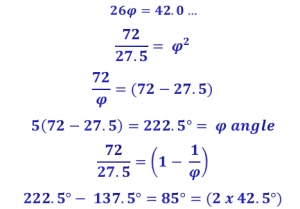Moreover, 72 is both the Aspect of the YHVH (יּוד־הי־ויו־הי) at the level of Chochma, and the square or radiance of the YHVH (יהוה): (י־יה־יהו־יהוה).

And while 42.5o connects to the value (425) of the 8th of the 72 Triplets (כהת), the total number of occurrences of the Torah’s 4th, 2nd and 5th words is 2701, as in the value of the Torah first verse (2701), the 73rd Triangular Number.

Meanwhile, the total number of occurrences of the Torah’s 4th and 2nd words is 2628, as in the value of the 72nd Triangular Number.  Since the sum of the 22 Names of the Alef-bet equals 4248, the difference between them and the 72nd Triangular Number is (42482628) = 1620, as in very nearly Phi (1.618), and as in the chart of the 72 arithmetic progressions from 18. This is also the sum of the products of the coordinates of the 72 Triplets.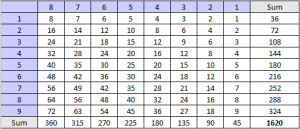The value 1620 is also 1/4th the square root of 42, and the complete sum of the Names of the 6 Matriarchs—Sarah, Leah, Rivka, Eve, Miriam, and Rachel—(505, 36, 307, 19, 290, and 238) plus their ordinal values (46, 18, 46, 19, 56 and 40) = 1620, which are all found in the 42-Letter Name Matrix.

These are not numbers for number’s sake. The Creator does not make His decisions based on numbers or mathematics. Mathematics and the resultant Numbers happen because of the Creator’s decisions. The idea is not to look for the Creator in a sea of numbers and infinitely possible equations. All of them belong to the Creator and all were set into motion through a cavalcade of cascading decisions or networks. Most people see only the end results, not the process. They see the paint color on the physical walls, not the Sheetrock, plumbing, wiring, framing, or how the wood, wires, pipes, plaster, paint were made, or where the minerals came from in the earth, in the Sun, in the universe, into existence.  Trillions of coincidences had to align just right for us to get the right shade of eggshell white on our walls, just in time for the back of a chair to scuff them up.

Do not see the Creator in the sea of numbers; see the sea of numbers as the Creator, and find the rivers that flowed into it, then find its tributaries, and streams, follow the valleys and climb the mountains from which they flowed. Each traces a journey of planned, tested, and well-thought-out branched decisions made by the Creator, a series of specific equations and networked numbers that lead back to the Source.

The number 72 is not important because of the 72 Triplets (Names); that important set of Triplets and letters and values coalesced around the number 72. It is one of those valleys or mountains from which an ever-widening set of cascading networks emerged. And thanks to the fractal nature of the universe, the Creator’s Creation, the essence of those numbers or emanations are still visible in the paint on the walls. The writing is on the wall. Where we see eggshell white, a higher consciousness sees thousands of nuanced colors and shades and shapes. It is like sitting on your couch with your eyes closed after watching TV or sitting on it after taking DMT. Your physical position does not change but your spatial one is completely different.

Quantum mechanics tells us that that wall is not there unless we look at it, and that it is the observation and/or interaction with it that makes it a wall. It can be a physical boundary, a metaphor, or a transcendent portal into the wonders of hyperspace dynamics where what we call physicality no longer exists.

The Creator did not start with the paint can, and neither do we. Because of the fractal nature of the universe, some of us can pick up brushes and turn paint into a creation, some into masterpieces, creating whole new worlds, a whole new set of descendant cascading networks.

In the allegory of the Tree-of-Life, Man chose the paint over the portal, the fruit over the transcendence. We can descend fractally lower or climb fractally higher. Either way it is a process. The paradox is that the shorter journey is the one less traveled in contrast to what most of us were taught. The beauty is that once the ascendant journey has begun the pathways are illuminated.

So no, we do not follow the numbers and equations or cosmic relationships to prove the existence of the Creator, we follow them to better Understand the Creator, to get closer to His process and reintegrate our consciousness with it. Simple fact: There is no creation of any sort on any level without a creator. Once we accept existence, we must accept a Creator. The question is are we satisfied with one painting, one book, one movie, one story, one lifetime, or do we want to see and experience it all.

### The Sun and the Moon and the Tower of Truth

As a virtual phenomenon, the Tower of Truth—so named because it has a volume of 44,100 cu3, and 441 is the value of the Hebrew word Emet (אמת) meaning truth—is metaphysically spectacular, yet it actually exists in stone and has been preserved for us for thousands and thousands of years, but more about that in a separate upcoming article.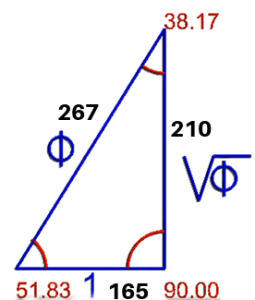Meanwhile, every cubic cubit (1 cubit3) of stone in that monument and in the walls and buildings of the Holy Temples equaled 12.0… cubic feet. This aligned them with the anomalistic cycle of the Moon and its balance between the Earth and the lunar calendar from which all our holidays or cosmic windows are based.  Since the anomalistic year works out to 12.0085 months, the Event Horizon radius of 5778 years in terms of the anomalistic cycle is 5782.10 years long, and the Spiritual Time radius of 3760 years would be 3762.67 years, an additional 2.67 years. While 210 cubits is the height of the Tower of Truth, 267 cubits is the apothem or hypotenuse of that tower when converted into a monumental right (90o) triangle based on the values 72 and 42.

This mysteriously aligns with the sum of the 90 doubled words in the Torah that is 26705 and with the square root of the total ordinal value of all the letters Zadi (צ) in the Torah, 267.050, especially since its letter value is 90.

What is amazing is that the hypotenuse of the Tower of Truth, 267 cu, divided by its base 165 cu, equals Phi (φ), 1.618…. and since the slope angle, or angle of ascent, is 722 or 51.84o its complementary angle of descent is almost exactly 1/φ2.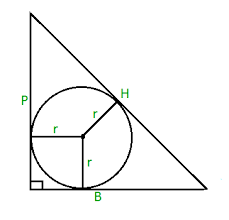Given the 722 or 51.84o angle of ascent and simple geometry, the radius of a circle drawn to neatly fit inside that vertical triangle (165210267) is 54, as in the 54 portions in the Torah, the 54-unit shift that aligns the 1111 Odd/Even split in the Alef-bet with Phi (φ), and the 72 Triplet Triplets whose two sets of square roots summed to 1000.054.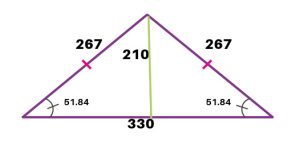If instead of just forming a right triangle of 210 cubits high, 165 cubits as the base, and 267 cubits as the hypotenuse, the Tower of Truth formed an isosceles triangle that was still the height of 210 cubits and with the same the slope angle, the 267 cubits length for the sides (hypotenuse) would stay the same and the 165-cu base would double to 330. Given those measurements, its perimeter would increase from (210 + 165 + 267) = 642 cu to (330 + 267 + 267) = 864 cu, matching the diameter of the Sun (864,000 miles). We could call this the Sun Triangle.  We also note that the difference between the two Triangles is (864642) = 222, as in (60005778) = 222 and the 222.5o Phi (φ) angle.

It is noteworthy that the 165 cu and 330 cu respective base measures of the two Tower and Sun Triangles reflect an exact .333 and .666 split in the 495o turned in the Spherical Time paradigm every 10 seconds; and the 495 sum of the 10 remainders 36, 72, 90, 45, 81, 18, 54, 09, 27 and 63 from dividing by (27.5); and the sum of the 3 x 3, 6 x 6, and 9 x 9 normal Magic Squares (15 + 111 + 369) = 495; and the sum of the 18 values of the Hebrew letters from Alef (א) to Zadi (צ).

### The Sun Triangle

If instead, the 267-cu hypotenuse formed an equilateral triangle its height would be 231 cu as in the 231 Gates of Wisdom.

Nonetheless, the 267th Triangular Number is 35778, as in the 3 occurrences of the Spherical Time radius …5778… within the first 1000 digits in Pi (π).

And while the square roots of the 72 Triplets and its 8 row totals sum to 1000.054, the square roots of each of the 9 column totals add up to 267.7.While we know that each of those 864 cubits of the isosceles triangle measures 27.5 in, if instead, we divide 864 by the Primal Frequency 27.5, we get 314.18 or 314.2, as in Pi (π), and as in the 3142 of the High Holy Names Ehyeh Asher Ehyeh (אהיה־אשר־אהיה) and the combined two sets of the spelled-out Names YHVH (יהוה) and Ehyeh (אהיה).  In hyperspace and metaphysics numbers have their own meaning and are not limited by whatever measuring modifier we attach to them so we can just as easily multiply 864 by 27.5 as divide by it. It is the similarity of form that we are looking for, not an Alltrails map to traverse the Sun.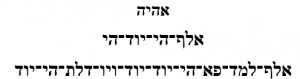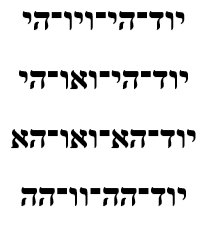If we did measure out those 864 cubits of the perimeter of the Sun Triangle in inches we would get (864 x 27.5”) = 23,760 inches = (18,000 + 5760 HC), as in the year 2000 CE, which is interesting because the angle 18o equals .3142 radians.

Moreover those 23,760 perimeter inches of the Sun Triangle divided by 3, as an average of the 3 sides, equates to 7920, as in the 7920-mile diameter of the Earth.

And when we divide that Sun Triangle 23,760-inch perimeter by the 5280 ft mile, we get exactly 4.5, the gematria of Man (45).

Those 23,760 inches are also 1980 feet, which is 660 yards and is 37.5% of a mile. This also means that those 23,760 inches divided by 123, a cubic foot, equal 13.75 cubic feet, which aligns the Sun Triangle with not only the diameter of the Earth but with the Phi (φ) angle (137.5o) and the rod of 6 cubits.

Those (864 x 27.5”) that equal 23,760 inches in the perimeter of the Sun Triangle also break down to 20,000 plus 3760, as in the 20 levels of the Tower of Truth on which it is based and as in the Spiritual Time radius 3760 years.

As for the radius of the Sun, 432,000 miles, it is related to the 432 Hz frequency of Binah hidden in the first and last word of the Torah cycle as previous explained, and 432 Hz/27.5 Hz = 15.71 which is naturally going to be Pi (π)/2 but 1571 is also the 248th Prime Number, as in the 248,000φ elements in the Torah.The square root of the area (34650 cu2) of the Triangle of the Sun is 186.145, which is exceeding close to the speed of light (186,282 mps), off by .0735%.  And since the twin slope angles, or angles of ascent, are each 722 or 51.84o their complementary angle of descent is 76.32o or 2/φ2, which is .212 of a circle (360o), as in half of 424 and as in the gematria of “The Light (האור),” appropriate for the Triangle of the Sun that incorporates the speed-of-light.

### The Sun Triangle and the Earth’s Circumference

The Tower of Truth and thus the Sun Triangle is 20 stages of sequential cubes (13 + 23 + 33203), giving rise to its 210-cubit height. The Earth has a circumference of 2πr or 2π(3960 mi) = 24881 miles and using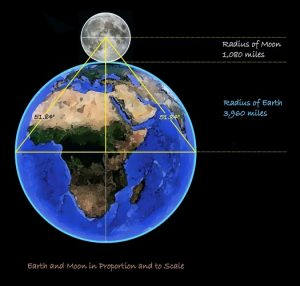Pythagoras’ theorem we can determine that for every 1 mile of curvature, the Earth’s surface drops away .66666666 ft, which is 8 inches. This is not about flat earth because in a Divine simulation it does not matter one way or another; it is just a perceived projection and from the perspective of the higher dimensions it is all flat anyway. No, this is about those 8 inches of descent per mile2 over 20 miles being equivalent to (202 * .6666666) ft = 267 feet, as in the 267-cubit apothem or hypotenuse descending from summit of the 20-stage tall Tower of Truth and the Sun Triangle.  Thus, using the few given figures for the Sun Triangle and simple geometry we can reverse engineer the circumference of the Earth.

One cannot help but wonder though about why the Earth’s curvature is .66666666 ft/mi and the Earth travels around the Sun at 66,670 mph, and the Earth’s tilt angle is 66.6o to the equator?

The complementary angle to 72o is 18o. If we add the 183 cube or 5832 verses in the Torah to the 378 or 33 Essential Magic Cube of Creation, we get 6210, which is 1/30th the speed of light (186,282 mps), off by only 18 mps. Thus, the speed of light is 30(183 + 378) – 18 mps, and (186,2826210) = 180072.

### The Shorter Cubit and the Meter

That 37.5% of a mile is interesting and we will understand it better as we get into the Prime Frequency of 27.5 and its relationship to Phi (φ) and the circle. It is interesting for another reason though. There is another measure for the ancient cubit that has been floating around for centuries based on speculation about the length of a man’s arm and that it comes up in some ancient structures. That measure is 20.625,” but this is just 75% or ¾ of 27.5 inches so it is understandable that it would appear.

By simple arithmetic, 4 of those short cubits equal 3 sacred cosmically and mathematically based ones, like the 4πr2/3 formula for the volume of a sphere.  Nonetheless, so-called archeologists use the result of dividing 20.625 inches by the ratio φ2/5 to get a figure of 39.390756 inches, which is very close to the measure of 1 meter, 39.3701 inches. To them, this suggests a knowledge and worse yet, a usage of the meter in ancient structures. While this is exceeding close to the meter, off by only .0512% what they do not realize is that it is entirely predicated on the 27.5” cubit and to Phi (φ) with which we know it is integrated.  Those 39.390756 inches are 3.75cu/φ2 and thus must somehow be related to the Sun Triangle’s 37.5% of mile perimeter.

The end result is that by multiplying a measure in meters by .6982 we can get a very close approximation to that same dimension in cubits. Once we do that we can thus determine whether all those ancient structures that have only been catalogued in meters were actually part of a network of megalithic construction. If so, then this prehistoric network would have been based on an intimate knowledge of the mathematical language of the cosmos, like the Triangle of the Sun.

Earlier we discerned that there was a relationship between φ2/5 and π/6 that were nearly identical (.523608) and that they related to the volume of a sphere (4πr2/3), particularly the volume of a sphere inscribed in a cube. We just saw that based on the cubit of 27.5 inches, the 3/4 cubit is φ2/5 or .523608 of a meter, which means the ratio of our ¾ cubit to the meter is the ratio of the volume of a sphere inscribed in a cube.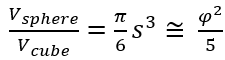This gives us a geometric proportion that we can apply to any given figure in meters or kilometers, like the distance between the Earth and the Moon. It is 384,400 kilometers aligning it to the value of Keter squared (6202) and to the 384,400-value of the 4805 letters Pe (פ) in the Torah. For whatever reason this works out to 201,273 of our ¾ cubit times 1000 for the kilometer. That 201,273 is exactly 200,000 less that the 5 main elements of the Torah (401,273). The Cosmos have been designed so that the ¾ cubit would give it extra richness and dimension.

Knowing all this it becomes apparent that the relationship between Pi (π) and Phi (φ) is φ2/5 = π/6 and thus their ratio is φ2/π = 5/6, and conversely π/φ2 = 6/5 = 1.2. This ratio is off by only .00001276. It also shows us the Time Coefficient (12) is derivative of the ratio or interactions of the Pi (π) and Phi (φ) fields.

Nonetheless, like the shorter ¾ cubit, ¾ of the Sun’s 864,000-mile Diameter or ¾ of the 864 perimeter of the Sun Triangle is 648000, as in the 6.480 square root of 42. And those 39.3701 inches in 1 meter, include the value (3701) of the 42-Letter Name, so regardless how they came up with the measure of the meter a couple hundred years ago, it is nothing without the inch and the cubit to integrate it with Phi (φ), Pi (π), and the Primal Frequency (27.5 Hz).

It always comes back to the Source (42). And ¾ of the Earth and Moon Diameters is 7560 or (10 x 27.52), the radiance of the Primal Frequency.

### Cosmic Circles in Time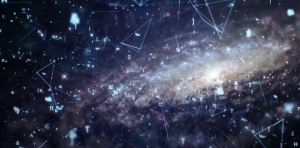With the Cosmos and Solar System all aligned in a cohesive pattern, and the network that binds and scaffolds that pattern more apparent the deeper we explore, we must ask if the Time component or constant in that pattern, 12, is not simply designed to be the ratio of π/φ or with a shifting decimal place 10π/φ, giving new meaning and understanding to the 12 lunar months and 12 arcs.

If this ratio were the circumference (c = 2πr) of a circle of 12 units around, the radius would be 5/φ and the area of that circle (a = πr2) would be 30.000, giving new meaning to the structure of the letter Lamed (ל) whose value is 30 and whose ordinal value is 12. We know the structure of the Hebrew letters were cosmically designed and are substantially based on Phi (φ), so does the letter Lamed (ל) represent the area and circumference of a circle, and in particular one of radius (5/φ). And since the complete value of the Lamed (ל) is (30 + 12) = 42, we can assume the Source Singularity of 42 plays a role in this as well. Moreover, there are 21,570 letters Lamed (ל) in the Torah or exactly 30 less than 21600, as in the diameter of the Moon (2160) and the 216 letters in the 72 Triplets. It also means the total value of those Lameds (ל) is 302 less than 648,000 as in the square root of 42, and the numerous connections of 6480 with the Torah elements.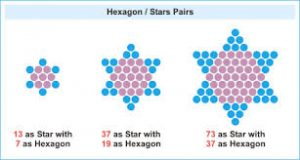There are 12 months in lunar calendar that guides the Cosmic Calendar in which all our holidays or cosmic opportunities are based upon. It order to reconcile the lunar and solar calendars a 13th month is added in 7 of every 19 years that mimics the inner and outer balancing act of the centered hexagram and the Magen David, which as we will see in an upcoming article is aligned with our DNA.

When the 1495-value of the Alef-bet was used as the area of a circle and split into its Odd/Even proportions, it took a shift of exactly 13o to align it with the Phi (φ) angle proportions.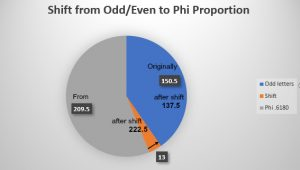The radius of that 1495 area Alef-bet circle is 21.81… and using the Time Constant, 12/21.81 = .5500020625, showing us its relationship to 55 or twice the Primal Frequency cycles (2 x 27.5) and to 20.625, our ¾ cubit and link to the meter. As the whole number 22, it is cosmically fitting that the 22 Letters of the Alef-bet have an area of 1495. This is yet another element in the design of the Alef-bet and adds yet another layer of beauty and dimension to it.

The value 21.81 of the Alef-bet radius comes up again in the ratio of the Spherical Time diameter and the cycles of 27.5 Hz, 11556/27.5 Hz = 420.2181, along with the Source Singularity of 42.

Nevertheless, given the relationship between the Time Constant (12) and the Primal Frequency (27.5) it is cosmically appropriate that the regressive summation series of 27.5 that equals 30 is directly aligned with the area (30.000) of the circle of circumference 12 or 10(π/φ2).  Moreover, 12 cycles of 27.5 Hz equal 330 or 11 times that area of 30, referencing the 11 beat structure of dividing by (27.5) that is based on the 10 cycles of 9. So it is also appropriate that the diameter of that circle of circumference 12 is 3.819 or 1/10th the complementary descent angle (38.16o) to the slope angle (51.84o) in the Tower of Truth Triangle.

### 11 Sefirot Aligned with 42 and 27.5Oddly enough, while one cubic cubit (1 cu3) of stone has a volume of 27.53 cubic inches, which is reminiscent of the 2753 value for the 39 letters in the backs of the Names of the 22 Hebrew letters, a sphere inscribed in any of those 1 cubit3 blocks of stone would have a volume of (10 x 332) cubic inches.

For whatever reason a sphere inscribed in a 1 cubit3 cube matches the 332 or 1089 initial Gimmels (ג) and Dalets (ד) in the Torah. Given the 11 sefirot (dimension) structure of the universe and the 11-interval nature of the Primal Frequency, it is notable that this equal (110011), or 27.5 x (40 – .4).

Earlier, we wrote about the relationship of the series of the set of odd numbers to the volume of a Sphere and the Cosmic Harmonic, and about the series of the set of perfect square numbers to the relationship between a sphere inscribed in a cube. We also then equated that ratio to φ2/5 so it feels natural that a sphere inscribed in a 1 cubit3 cube should work out to a perfect square (332) tied to the Primal Frequency that is also tied to Phi (φ).

Another odd cosmic alignment is found in the first and last letters (כת) of the 42 letters of the 11 sefirot that sum to 3342, which itself forms the simple equation (3300 + 42) = (27.5 x 120) + 42. The letters Caf (כ) and Tav (ת), which according to Abraham Avinu represent the Sun and the Moon, have a combined numerical value of 420 and an ordinal value of 33, as in 3342.

This is like the number of paragraphs in the Torah’s first and last Books that total (91 + 159) = 250, leaving exactly 420 in the center 3 Books.

Perhaps it is not odd at all, considering that all 11 of their initials total (20 x 27.5), as in the 20 sequential stages of the Tower of Truth, with the lowest stage/level being 20 cubits high and having a base area of 400 square cubits, just like the Sun (20) and the Moon (400), whose physical size ratio is 864000/2160 = 400, the same ratio as the 203 cubic volume of that 20th stage to its height, 8000/20 = 400 cubits. It could be said that the foundation stone of the Tower of Truth contains the Solar/Lunar ratio while the full Triangle of the Sun contains the Solar/Terrestrial ratio.What is even more metaphysically intriguing is that while the 432,571 sum of the 42 products (1 x 20 + 2 x 400 + 3 x 200…400 x 400) of the 42 Letters of those 11 sefirot (dimensions) paired with their 42 counterparts in the 42-Letter Name hints at the Sun’s radius (432,000 mi), the sum of their 42 square roots equals (220022), or 2(110011) which equals 2(1089) = 2(332).

Meanwhile the P/S cipher for those same combined 42 Letters of the 11 sefirot (concentric spheres) paired with their 42 counterparts in the 42-Letter Name is 888.0, or (8 x 111.0).

Moreover, the 42 square roots of the 42 sums of the 42 Letters of those 11 sefirot (dimensions) paired with their 42 counterparts in the 42-Letter Name when using the gematria albam cipher that is based on the number 11 equals 424.805, as in Moshiach Ben David, the Cosmic Consciousness, and as in 4248, the sum of the 22 Names of the Alef-bet, and as in the 4805 letters Pe (פ) in the Torah.

### The 20 Stages of the Tower of Truth.

The Tower of Truth and thus the Sun Triangle is 20 stages of sequential stacked cubes (13 + 23 + 33203), giving rise to its 210-cubit height. Over those 20 stages or 20 stacked cubes it has a volume of 44,100 cu3, and since 441 is the value of the Hebrew word Emet (אמת) meaning truth, hence the name “Tower of Truth.”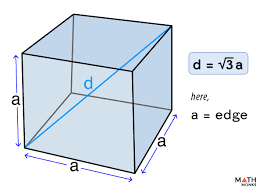Each one of those 20 blocks or cubes has a diagonal equal to the square root of 3 or (1.732…) times its side length. While the square root of 3 or (1.732…) also reflects the 173-value of the 42-Letter Name, the sum of the 20 diagonals is 2103 = 363.73067, as in the H’Moshiach, the Cosmic Consciousness, and the numerical values of the 2 highest sefirot (dimensions) attainable, Chochma (73) and Binah (67).

While the Tower of Truth shares the same total 2103 = 363.73067-cubit length for its diagonal sum with a solid cube of 210-cu per side, the volume of the Tower of Truth is exactly 1/210th that of that solid 2103 cu cube.

### Block by Block

Given the ratio of the sphere inscribed in a 1 cubit3 cube, it is not surprising that the word sefirot means “spheres.”

What makes perfect sense metaphysically, yet is nonetheless surprising, is that the standard gematria of the word “sefirot (ספירות)meaning dimensions or spheres, is 756, as in the radiated Primal Frequency, 27.52 and as in the base measure of the Sun Triangle in feet (756 ft). The ordinal value of “sefirot (ספירות) is 90, giving it a complete value with the kolel (2) of 848, or (2 x 424).In Genesis 11:1 it says, “The entire Earth had one language with uniform words.” Two verses later it says, “They had bricks to use as stone and mortar.” The numerical value of the word used for mortar, chemar (חמר) is 248, while the word for bricks, l’venim (לבנים) has a complete value with the kolel of 756, as in “sefirot (ספירות) and of the area of a cubit, which is the same as the radiated Primal Frequency, 27.52 and as the base measure of the Sun Triangle in feet (756 ft).

The word brick l’venah (לבנה) is the same as the Hebrew for the Moon l’vanah (לבנה) and comes from the word white lavan (לבנ), which is also the name (Laban) of a forefather of a long line of evil vengeful sorcerers.  At the very least, we can extrapolate that the bricks were white.

This 32nd paragraph in the Torah, once again hints at the great reset, but we will cover that in another article, except we need to ask why it says, “Let us make ourselves a name so that we will not be scattered all over the face of the earth.” How did they know G-d would scatter them before it happened? As we have seen, the Torah is extremely deliberate and there are no semantic of chronological mistakes as some people would lead us to believe—everything is in just the right place and serves numerous purposes. They were worried because they knew it had happened before. The Zohar tells us that their purpose in building the Tower to the sky (Heaven) was to rearrange the sefirot and establish a New World Order, a One World where they would control everything by placing physicality (Malchut) above Keter, quite similar to our world today.

While a stone or brick cube formed with 3 cubits3 in volume has an inscribed sphere equal to precisely Pi (π)/2, a cube formed of (3 x 27.5”) in cubic volume has an inscribed sphere of 43.2” cubic inches, as in the radius of the Sun, 432,000 miles.  Doubling that to form a cube of (6 x 27.5”) in cubic volume, as in the length of the measuring rod used for the Holy Temples, gives us an inscribed sphere of 86.4” cubed, as in the 864,000 mi diameter of the Sun once again.

The 10th Triangular Number and 10th Fibonacci Number is 55 or (2 x 27.5) while the all-inclusive or Tetrahedral Number for the 10th Triangular Number is (55 + 220) = 275, as in the measure of the cubit (27.5”), or more precisely the measure of 10 cubits, just like the height of the beams in the Mishkan (Tabernacle) of 42 elements. All 3 of those values(55 , 220, 275) are found among the 72 Triplets,  the 48th, 29th and 39th respectively, as are the values 20 and 330 for the 62nd and 60th Triplets. Also 42 multiple times and in multiple ways and 210 in the very center, reinforcing the connection between the 72 Names (Triplets) and the Sun Triangle. Moreover, the word cubit, Me’ah (מאה), has a numerical value of 46, as in the 65th Triplet of the 72.

This is rarer than we might think because each of those Triplets could have a value anywhere between 3 and 1200 and those values could repeat, making it only a .0835% chance that a particular number will appear in any given box and a 6.01% chance that one will appear among the 72. The odds get worse and worse for each subsequent value that we need to find. We must also keep in mind that these 72 Triplets unwind in sequential order to form the 3 coherent 72 letter verses at Exodus 14:19 at the exact moment of the Splitting of the Red Sea.

The 10th stage or cube thus starts 55 cubits from the Top of the Tower and 155 cubits from the bottom and thus the numerical top half represents exactly Phi (φ)2 percent of the Tower. Through the 10th stage or cube the cumulative area covered by the 10 cubes is 385 or (12 + 22 +…102) = 385, representing the Shechinah or Divine Presence of the Creator. And the cumulative area covered through the 5th Cube is (12 + 22 +…52) = 55.

### Pyramid of the Sun

Meanwhile, a square of 2 cubits per side has an area of exactly 552 or (27.5” x 2)2 = 3025 in2, and 30252 equals the distance between the Earth and Sun at their closest point. It is just a matter of scaling up or projecting downward as happens between the hyperspace dimensions.

The 8-cubit perimeter of the 3025 in2 square aligns with the 842 or 722 slope angle (51.84o) of the Tower of Truth and the Sun Triangle.

Since the Sun Triangle gives us a base of 330 cubits, that base can serve as an edge of a platform or square of area 3302 cu2, which is also 756.252 ft2, like the value of the Hebrew word sefirot (ספירות), meaning spheres and dimensions.

Its perimeter is thus (4 x 756.25 x 12”) = 36,300 inches, as in the Spherical Time circumference 36304.24470 or 36300 + 4.24470…, which represents H’Moshiach and Moshiach Ben David, a double dose of Cosmic Consciousness. In feet that perimeter is 3025 ft, and in cubits it is 1320 cubits, as in 10 times the standard value of the Hebrew word for bricks, l’venim (לבנים) that is also associated with 756 and 27.52.

This also means each side of the Sun Triangle platform base would be 27.52 ft and the base area would be 27.54 ft2. Since 27.5 is 1 cycle of the Primal Frequency 27.54 ft2 is metaphysically equivalent to 14 cycles, an expansion of One that has no relevance in a physical world and can only be understood with hyper dimensions.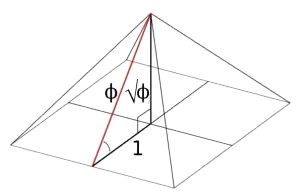Once that hypothetical platform is laid-out and the Sun Triangle is duplicated perpendicularly, a perfect 4-sided Sun Pyramid could be formed, with all the same angles and attributes plus the additional attributes of having corner slope angles of 42o and face to face edge angles of 112o, plus the apex angle of 76o, as in the ordinal value of the Torah first word; and not to mention the ratios and dimensions of the Earth, Moon, and Sun.

While the platform forms a perfectly square base of 27.54 ft2 with a 3025 ft perimeter, it is also 42 x 72, metaphysically representing the merging of the 14 Essential Triplets of the 42 Letter Name with the 72 Essential Triplets, just as the slope angle (51.84o) does.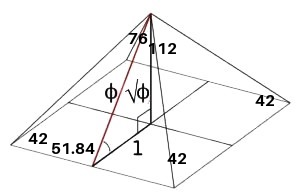And since 27.54 ft2 is metaphysically equivalent to 14 cycles, those two merged sets of Essential Triplets, representing the 114 human avatar chakras (112 corporal and 2 extracorporeal) are also equivalent to 14 cycles. This in line with the 72 verses in the first 9 paragraphs in the Torah and the 9 rows in the 72 Triplet Matrix from the Torah, and the subsequent 42 additional verses through chapter 14 for a total of the first 114 verses in the Torah, once again linking the 72 Essential Triplets with the 14 Essential Triplets of the 42 Letter Name, as we see linked in the Pyramid of the Sun.

Then because there are 12 inches in every foot, the area of that platform is also physically 122 x 27.54 in2 or metaphysically 122 x 14 cycles.  In terms of cubits, it is 122 x 27.52 cu2, essentially the same as in inches (122 x 14 cycles), or alternatively 33 x 120 cycles which is also 332 x 102, possibly reflecting the 33 letters in the 11 Triplets of Creation.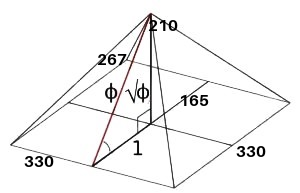Using the simplest of geometry, we started with the 210 cu height given by the Tower of Truth, the Primal Frequency 27.5, and the Phi (φ) proportion, and created the Cosmic blueprints for a perfect pyramid that incorporates the 72 Triplets, through the slope 722 angle; the 14 Triplets of the 42-Letter Name through the corner slope angle (42o); the 11 Triplets or 33 letters of Bereshit through its apex angle of 76o and base edge of 330 cu; and the full 112 Triplets through its face to face angle (112o) that in turn align with the 112 chakras of the human body or avatar.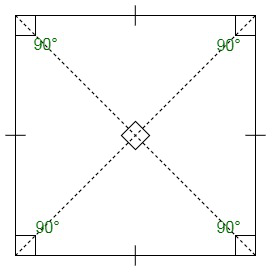Each of the 4 edge lengths of this Sun Pyramid works out to within 2 inches of 314 cubits or about 720 ft. The vectors from the central base point of the Tower of Truth to the 4 corners of the Sun Pyramid, in other words the 4 semi-diagonals each measure 233.345 cubits, as in Moses (345) and the unified sum (233) of the 2 expanded spelled-out Names YHVH (יוד־הי־ויו־הי) and Ehyeh (אלף־הי־יוד־הי), and as in the ordinal values of the 18 letters of the outer 2 tiers of the Alef-bet, (45 + 188) = 233, which may be entirely coincidental except that the full 3 tiers of the expanded spelled-out Names YHVH (יהוה) and Ehyeh (אהיה) do equal 3142, and this Sun Pyramid is being created entirely out of the unfolding geometry of the Creator and the Cosmos.

Those 233.345 cu semi-diagonals of the Sun Pyramid’s square base also each measure exactly 27.572 cu, and the full diagonals are thus 5572 cu, once again emphasizing the design connection to the Primal Frequency (27.5) and the 72 Triplets, just as the height of the Sun Pyramid and Tower of Truth is (5 x 42) cu as in the 42-Letter Name. In inches those semi-diagonals thus become 27.52(√72). So, the coefficient for 42 in the height of the Tower and Sun Pyramid is 5 and the coefficient for 72 in the base of the Sun Pyramid is 27.5, while the ratio between them is 27.5/5 = 5.5 or (2 x 2.75).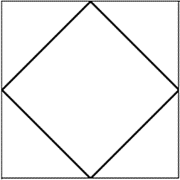Those 4 semi-diagonals of 27.572 cu have equal counterparts that connect the 4 hypotenuse points or apothems of the Sun Triangles and of the original Tower of Truth Triangles, whose slope angles were 722. Because of that slope angle, every 1/72 of rise in the Tower is equivalent to a rise of about 6.666666 feet, similar to the curvature of the Earth. The square formed by the 4 semi-diagonals of 27.572 cu is equal to half the base platform of the Sun Pyramid, as are the 4 corner triangles, and a circle drawn around the 4 contact points would be inscribed within the base. That inscribed circle’s perimeter is 11072, which is equal to the sum of the diagonals of the entire Sun Pyramid.

The square root of 72 or √72 is an interesting irrational number, 8.48528137424…. since 848 is: twice 424, Moshiach Ben David; the mile is 5280 feet; the Fine Structure Constant is 137; and 424 is Moshiach Ben David again, the Cosmic Consciousness.The value 137 is also kabbalah, meaning “to receive” and parallel.

Meanwhile. each of the resultant corner triangles has an area of 13,612.5 or 495 x 27.5, as in the 3 x 3, 6 x 6, and 9 x 9 normal magic squares that equal (15 + 111 + 369) = 495; and as in the sum of the 10 remainders 36, 72, 90, 45, 81, 18, 54, 09, 27 and 63 from dividing by (27.5) that equal 495; as in the sum of the 18 values of the Hebrew letters from Alef (א) to Zadi (צ); and as in the 495o turned in the Spherical Time paradigm every 10 seconds.The area of this inner square that is exactly half the Sun Pyramid base is 54450 cu2 or (55,000550) cu2 = (1980 x 27.5) cu2, which is exactly 72 x (27.52). This means that this inner square, which is at a right angle to the orientation of the Sun Pyramid, is comprised of precisely 72 squares of 27.5 by 27.5, the sacred cubit times the Primal Frequency. This is akin to the 72 squares or positions in the 72 Triplet Matrix, and thus inserting the radiating Primal Frequency through each of the 72 Triplets. It gives us new insight into the Cosmic design of the Pyramid of the Sun and of the 72 Triplets.

The 5845 verses in the Torah can be split into 5500 and 345 or (200 x 27.5) plus 345, the numerical value of Moshe (משה), and the 5th Triplet (מהש) of the 72 Triplets, as in the 5 Books of Moses.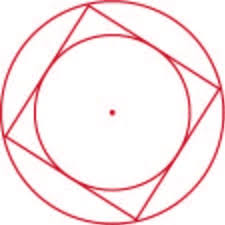The ratio of the area of the Sun Pyramid’s complete square base to the area of the inscribed circle in it is the Cosmic Harmonic (1.273). And while the ratio of the circumscribed circle using that semi-diagonal as a radius is Pi (π)/2, the ratio of that circumscribed circle to the inscribed one is exactly 2, like the ratio of the inner square (72 x (27.52) cu2) to the full base square (122 x (27.52) cu2) or 27.54 in2.

As for the ratios of their circumferences. The ratio of the square base perimeter to its inscribed circle is also the Cosmic Harmonic (1.273), while the ratio of the circumscribed circle to the Sun Pyramid base is 1.1107, as in the Name Alef (111) and/or the 3 initials of the 3 tiers of the Alef-bet (איק), coupled with 107, as in the fated 107th Triangular Number, 5778. The circumference ratio of that circumscribed circle to the inscribed one is now exactly √2.

It is just simple geometry. Like the sum of the area in the base platform of the Sun Pyramid and its inscribed and circumscribed circles equaling 365489 cu2 which is exactly 233 cu, more than 365256, which represents the sidereal year (365.256 days).

There are 12 arcs of 3025 years or (4 x 27.52) per lunar arc in the Spherical Time Bubble or Cosmic Clock. Therefore, the Moon’s diameter of 2160 mi is to the Great Precession and Great Day (25,920), as 3025 is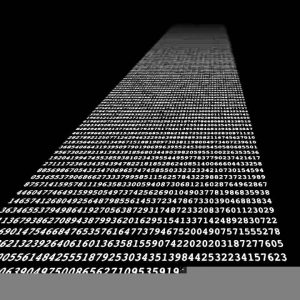to the Spherical Time Circumference (36304.24…).  The diameter of the Great Precession and Great Day (25,920) is 25920/π = 8250 = 300.0… cubits or cycles of 27.5 Hz, as in the ratio of the area of the base of the Sun Pyramid divided by its perimeter or 3302/1320 = 82.5 = 3 x 27.5. It is also like 5778/7 = 825.42 which is like the 210 cu height of the Tower of Truth and thus the Sun Triangle and Sun Pyramid in inches divided by 7 or 5775/7 = 825. And just like 5778 and 2019 being found 3 times in Pi’s (π) first 1000 digits, 8253 is one of the 42 4-digit strings found duplicated there.

Nevertheless, from the edge of the Event Horizon in 5778 HC, the last lunar arc was entered into 3025 years ago, in 2753 HC, 175 years before the construction of the First Holy Temple, aligning that date with the backs of the Names of the 22 Hebrew letters that equal 2753 and with the 3-6-9 split of the Alef-bet.  This date marked by the Spherical Time progression was in 1007 BCE about 137 years prior to the midpoint in 2889 HC.

Physical measurements are about tuning and alignments. Their source is in their frequency.  Time is a radiation in the aether; it is an effect, not a linear process, or a separate dimension.

### The Distribution of the YHVH (יהוה) and the Primal Frequency

Of the network of 26 x (27.5o + 42.5o) = 1820 YHVH (יהוה) in the Torah, 550 are in the 5th Book, as in the equations (20 x 27.5Hz) or (4 x 137.5o) that align with the collective height of the 11 Gates of the Holy Temple; the front letters of the 3 highest sefirot; the 11 initials of the 11 sefirot of the Tree-of-Life; and the 11 generations from Shem to Isaac. It is also most appropriately 10 times the sum of the integers from 110, and the sum of the 11 letters Nun (נ) or (11 x 50) = 550 in the 72 Triplets.

Meanwhile there are 165 YHVH (יהוה) in the 1st Book of the Torah, where we find “The Tree (הָעֵץ)” of numerical value 165, and the base of the Sun Triangle.  This makes the total YHVH (יהוה) for the 1st and 5th Books together equal to (26 x 27.5), which can be divided into their collective 84 or (42 x 2) Chapters.  There are then 3.333 times as many YHVH (יהוה) in the 5th Book as there are in the 1st, aligning and mimicking the ratio of the Sun to the Earth in terms of mass (333,000) and of the Sun to the Earth’s Great Precession (864,000/25,920 = 33.333), along with the 3.333 seconds in every part of a minute. The equation 864,000/25,920 = 33.333, or simply 100/3.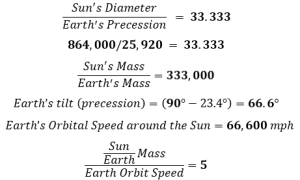Meanwhile, the 165 YHVH (יהוה) in the 50 Chapters of the 1st Book average 3.3 per Chapter, and the 550 YHVH (יהוה) in the 34 Chapters of the 5th Book average 16.18 per Chapter or Phi (φ). At this point nothing is neither odd nor a coincidence, like the 34 Chapters being the 9th Fibonacci Number and 55 being the 10th which explains why 550/34 = Phi (φ). It is also similarly relevant that the 34 Chapters of Devarim, the 5th Book, align with the 34 verses in the 7 Days of Creation to begin the 1st Book that are also proportioned as we recently saw into the Phi (φ) and Fibonacci proportions.

The number of YHVH (יהוה) in the 3 remaining Books of the Torah is thus (1820 – (550 + 165) = 1105 = (1100 + 5) = (40 x 27.5 + 5) YHVH (יהוה). It is also twice the 550 YHVH (יהוה) plus 5 that are in the 5th Book.

There are 26 x (27.5o + 42.5o) = 1820 YHVH (יהוה) in the Torah. To be in tune with the Torah means to be in tune with the 1820 YHVH (יהוה). This in turn means to be in tune with the Primal Frequency and Universe’s Heartbeat (27.5 Hz) and with the vibration of Moshiach or Cosmic Consciousness (42.5 or 42.489).

To be in tune with the Torah means to be on the Path of One represented by the 1820 YHVH (יהוה). It means to be aligned with the Love and Awe of the YHVH (יהוה).The equation (26 x (27.5 + 42.5)) aligns us with the Path of One and leads us to the Singularity (42). The total value of those 1820 YHVH (יהוה) that derive from the 424th Triangular Number of Yuds (י), Heis (ה), and Vavs (ו) in the Torah is (262 x (27.5 + 42.5)). This is the Radiance of Cosmic Radiation.

The ancient kabbalists explained squaring as the spreading out or radiating of the Name and thus the YHVH (יהוה) becomes YHVH (י־יה־יהו־יהוה) of numerical value 72.  When the squared value 72 replaces the 262 or YHVH (יהוה)2 in the equation (262 x (27.5 + 42.5)) we get (72 x 70) = 5040, which is the radii of the Earth and the Moon. The radii came from the radiance much as in physicality they came from the Sun. It is also 7! factorial as in the 7 dimensions that create physicality.

The full value of the 1820 YHVH (יהוה) in the Torah is 7!. When we use love and awe of the Creator and align ourselves with the Cosmos of His Creation we can become one with the 7 dimensions of Zeir Anpin, and not just a mere reflection.

The 10 Commandments begin with the 420th YHVH (יהוה) in the Torah, and when the Singularity at the heart of the core of Spherical Time and the universe beats 420 times, it means that (420 x 27.5) degrees have been meted out, 11,550 in total, like the 550-value of the 11 initials of the 11 sefirot (dimensions). This is the 20th Chapter in the Book of Shemot and the 70th overall and while (20 x 70) = 1400, there are exactly 1400 YHVH (יהוה) in the rest of the Torah, which is like the 420 Paragraphs in the central 3 Books of the Torah.

### The Circle and Cycle of Time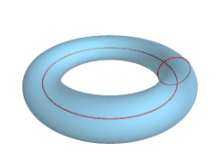It also means that the circle of time has been completely traversed, and the Spherical Time Event Horizon has been reached. At some point in that final 27.5o or 27.5-year period we reach the edge of the Torus, and everything cycles back toward the beginning of consciousness in our reflective world. This is why Man was created on the 6th Day, after the 6000 years of the back side or regression side of torus were completed. This is the midpoint in the galactic 12,000-year electro-current cycle, which lines up perfectly with the Heinrich extinction events and the Dansgaard-Oescheger oscillations in Earth’s history. And thanks to the interaction and ratios of the Primary fields of Pi (π) and Phi (φ) in the circles of Time φ2/π = 5/6, π/φ2 = 6/5 = 1.2, we can better understand the time aspect of the 12,000-year cycle.

The next cycle begins 27.5 years later at (11,550 + 27.5) = 11577.5 years, as in the (210 cu x 27.5”) = 5775” height of the Tower of Truth, and as in the 27.5 cycles/year in the 210 years in exile in Egypt (22382448 HC), understood as the realm of physicality. Those 210 cubits are about 480 feet—like the 6th and 9th magic squares (111 + 369)—in height and as in the 480 years from that exile in Egypt (2448 HC)—or (.424 x 5778)—to the construction of the First Holy Temple (2928 HC).

Since the First Holy Temple stood for 410 years until 3338 HC—or (.5778 x 5778)—it means that the entire period from entering Egypt to the destruction of the First Holy Temple lasted exactly 1100 years, as in (40 x 27.5), 40 cycles of 27.5 Hz. When extended to the full 430 years of exile, the total becomes (1100 + 220) = 1320, as in the perimeter of the base of the Pyramid of the Sun, or 48 cycles of 27.5 like the 48-story high Tower of Truth and Pyramid of the Sun. That 1320 year period began in 2018 HC, 3760 years before 5778 HC, the Western Calendar analogue to 2018 CE. Through this fixed Biblical and Cosmic dating we can begin to understand the interplay between the Spiritual Time and Physical Time radii and the Primal Frequency.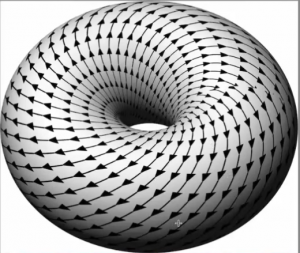As we enter the Event Horizon and approach the edge of the Spherical Time Torus time becomes stretchy and warped, leading to glitches in the simulation, Mandela effects, and unexplainable aberrations, such as mature 100 billion stars galaxies appearing in our telescopes where they should not exist, as if they were 13 billion years older than they should be.  Time is not dependent on the speed-of-light. What we perceive as the speed of light is dependent on time, and time is not linear.

This coincides with the absorption of the large X7 solar cloud into the supermassive black hole center of the Milky Way about 26,000 years ago—one complete great precession cycle—and the subsequent galactic super wave that fanned outward and will arrive here sometime in the next few years within this last stretched cycle of the Event Horizon when Earth’s magnetic field weakens to dangerous levels.

The first 42-Letters of the Torah that summed to 3842, and that form part of the 3842/1273 = 327.5 equation, have a complete value of 4273, further cementing their connection to the cosmic harmonic (273). It also further cements its connection to the 42-Letter Name Matrix whose complete value is 4225, which just so happens to be the complement to toroidal limit of the Event Horizon, 11577.5 years, since (422.5 + 11577.5) = 12,000, and to the Tower of Truth and Sun Pyramid in that (5775 + 4225) = 10,000.

As we saw earlier, the final cycle that ends at 11577.5 years contain the same numeric string 1577 that we found in the 9/.1230490273… = 73.14157771495 equation that was off from true Pi (π) by a miniscule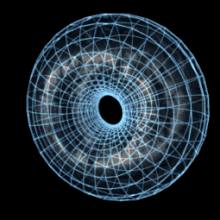amount and whose inverse was 2103, as in the 210 years of exile and as in the 210-cubit height of the Tower of Truth. It is therefore fitting that at the ultimate edge of our time Spherical Time toroidal bubble is the value 1577, the numerical value of the Ein Sof (אין־סוף), or the metaphysical Endless, the Infinite, G-d Transcendent, the Pure Essence of G-d, the state of the Creator before all Creation.The value 273 representing the Cosmic Harmonic (273) found throughout our physicality and universe and in the 42-Letter Name Matrix (1230490273) as 3 consecutive letters (רעש) of its second level that form the numeric string …273…. While their numerical value is 570 and their ordinal value is 57, they (רעש) permute, as explained to us by the Arizal, into the word Eser (עשר), the Hebrew word for ten (10), as in the 10 sefirot. The value 273 is also (13 x 21), the value of the higher Name Ehyeh (אהיה).

Another name for those 210 years represented in the Tower of Truth is “H’Har (ההר), The Mountain,” of numerical value 210. That word (ההר) is found 50 times in the Torah, as in the height of the 50 cu Gates of the Holy Temple that measure 1375” each and as in the 50 Gates of Binah. The Mountain (ההר) refers to Mt Sinai.

The Ein Sof (אין־סוף) on the other hand has a standard gematria of 207, like “Light, Ohr (אור)” and like the value of the word “B’Har (בהר)” that also refers to the Mountain. With the 3 prefixes (ומב) it is found 67 times in the Torah, as in the numerical value of Binah (בינה), the realm beyond our illusionary Spherical Time bubble.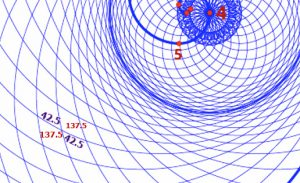The circle is the complete balanced cycle of 360o comprised of 2 passes through the Phi (φ) field and 2 through the Moshiach (Cosmic) Consciousness field: (2 x 137.5o) + (2 x 42.5o) = 360o. Any circle we draw in these dimensions will necessarily pass through these fields at least twice. While the wavelength of 27.5 Hz is fairly long the waves through the aether are pervasive as they condense in the 7 lower dimensions.

The circle, what we perceive as 360o, is “One” and as we know, the circle already has the Pi (π) field built into it. When the circle (One) is divided or filtered by the Phi (φ) field we get 360o /1.6180339887 = 222.5o and the balance, the completion of the circle, is (360o222.5O) = 137.5o. This is the portion known as “G-d’s Spirit,” the tip of the sword hovering over the face of the waters.

Then each half circle or (137.5o x 0.5)/1.6180339887 = 42.489 or 42.5o. This is the portion known as “The Light.”

While 137.5o divided by the Primal Frequency 27.5 is (137.5/27.5) = 5, the counterbalancing Phi (φ) angle 222.5o is (222.5/27.5) = 8.090909. Together, the two Primal Phi (φ) ratios are 13.090909 or One, echad, that is (360/27.5).

The portion of the circle of One that is “the Light” is thus twice (42.489 or 42.5) over 360, “the face of the waters” or (2 x 42.489)/360. It is exactly 1/φ3

About 3800 years ago, Abraham Avinu instructed us to place the 22 Hebrew letters in a circle and sort them into 231 pairs as the 231 Gates of Wisdom.  The value of those 22 Hebrew Names of the letters is 4248 and in pairs they would be (2 x 4248). Our language has evolved and we have different terms than the ancient kabbalists, but they saw what we are just now seeing.

### Cycles of Emanations

Since the radiation emanates and is measured in cycles of 27.5o and 137.5o we see that within the number 963 there are 35.0 cycles of 27.5o and 7.00 cycles of 27.5o making 42.0 cycles altogether, as in the Singularity of 42.

The value 963 is not only related to Tesla’s 3 6 9 but is also the value of the word Echad (אחד), One, when spelled out (אלף־חית־דלת). It is even more special than that though as it is the sum of the first 24 Prime Numbers, as in the 24 hours in One day.

Yet in relation to Spherical Time, 963 is both (9 x 107), as in the 107th Triangular Number, the Event Horizon radius, 5778, and exactly 1/6th of 5778, as in the 6 Lunar ellipses in the Cosmic Wheel. It is also 1/12 of the 12 lunar progressions of 963 years each in the Great Cycle of 11,556 years that matches the Spherical Time diameter.

While the 28th Prime Number, 107, is aligned with the 107th Triangular Number, 5778, which is the Spherical Time radius, the 28th Fibonacci Number is 11,556 cycles of 27.5o, the Spherical Time diameter (11,556 years).

The Torah sets aside 107 for the 13 verses of the 10 Commandments that begin at the 107,007 letter in the Torah, and much earlier as the 10 generations in the Book of Adam that begins with the 13th paragraph and the 107th verse in the Torah.  And just to be sure that there was no doubt in linking these two critical segments of the Torah, it is designed so that the 12th and previous chapter would begin with the 81st verse and contain 26 verses exactly like the first two words of the 10 Commandments that begin Anochi YHVH (אנכי־יהוה) “I am G-d” of numerical value (81 + 26) = 107.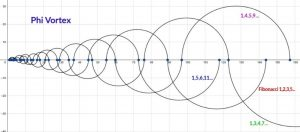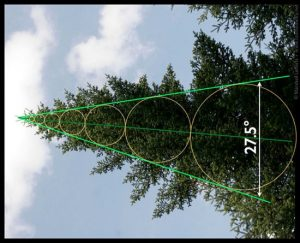It is by a function of the Fibonacci Numbers, which as previously explained form a vortex based on Phi (φ), that the cumulative value of the first 26 Fibonacci Numbers divided by 27.5 is also 11,556 or (2 x 5778). They correspond to the 26 generations of Adam through Moses that started at verse 107 in the Torah, and obviously to the YHVH (יהוה).

After resetting after the failure of the generations of Cain, the Torah began anew at verse 107, right after the final words in verse 4:26, “Then began men to call upon the Name of the Lord (לִקְרֹא בְּשֵׁם יְהוָה.)” whose initials equal 42 and whose middle word is the permuted acronym (שמב) for the 42-Letter Name, Shem Mem-Bet). This is like the portion Lech-Lecha, the 3rd in the Torah that begins the 42nd Chapter and 300th verse in the Torah for a total of 342, as in the value of Shem Mem-Bet (שמב).

To be clear 11,556 years is the diameter of Spherical Time equation and is equal to (12,000444), which is the value of “From Generation to Generation (לדר־ודר)” and as Rav Ashlag told me, 444/22 (letters) = 20.18181818, reflecting the year 2018 CE (5778 HC). In addition to that, 11,556/444 = 26.270270270…, which is reflective of the 26 generations through Moses, but what is more is that 11,556/42 (The Source) = 275.142857, the Prime Frequency and 1/7th.

The Creator saw things as “good (טוב)” of numerical value 17, seven (7) times in Creation and the value of the 7th Prime Number is 17, which equals 27.5/φ. That is enlightening unto itself, like the 7 times the higher Name Ehyeh (אהיה) appears in the Torah; nevertheless, there is no mathematical reason that the value of the 17 first cumulative or Triangular Prime Numbers should be exactly 440 or (16 x 27.5), 16 cycles of 27.5o, or that the first 7 cumulative Prime Numbers should equal 161, the highest aspect of the Binah Name Ehyeh (אלף־הי־יוד־הי). This Name numerically leads off Phi (1.6180…)  in the same way that the Name Shaddai (שדי) leads off Pi (3.14…).

Meanwhile, the sum of the 4th Triangular Number and the 4th Prime Number equals (10 + 7) = 17, and the sum of the 17th Triangular Number 153 and the 17th Prime Number, 59 equals 212, the numerical value of “The Light” or half of the 42.5o complementary angle to the emanating Phi (φ) angle.It is fitting then that 1533 equals 3581577, as in the consciousness of Moshiach (358) and the 1577 that we saw at the ultimate edge of our time Spherical Time toroidal bubble (11577.5) and in the value of the Ein Sof (אין־סוף), the metaphysical Endless, the Infinite and the Pure Essence of G-d.

Since 17 is the ratio of the Prime Frequency to Phi (φ), or 27.5/φ, it makes sense to examine the first 10 Triplets in Phi (φ). They total 6106, and the sum of the first 10 Triplets in Phi (φ) plus the first 10 Prime Number Triplets is (6106 + 4310) = 10,416, which is equivalent to the 42 rows times the 248 columns in the Torah Matrix, or (42 x 248) = 10,416. The two overlapping primal fields form the scaffolding matrix of the Torah.

### The Primal Cycle of the Torah and the Holy of Holies

The 58words, letters, verses in the Torah produce 58/27.5 = 14204.54… cycles of frequency 27.5 Hz. When we add the 42 Rows, 187 Chapters, 54 portions, 670 paragraphs and 22 letters, we get exactly 14240 cycles of frequency 27.5, as in 1424, the value of the Holy of Holies, H’Kodesh Kadoshim at the core of the Holy Temples.

While the 248 columns are equivalent to 9.01818.. cycles, the net 10,400 row-columns equals 378.1818 cycles of 27.5 Hz, as in the 378 ordinal total of the Alef-bet and Essential Magic Cube of Creation.

We should keep in mind that the Holy of Holies, H’Kodesh Kadoshim, measures a perfect cube of 203, the same as the foundation stage, the bottommost block of the Tower of Truth. It is also the same as the 64 or 82 Triplets that make up the foundation block or 8 Candles of the 72 Names (Triplets). This square block of 8 x 8 or 64 Triplets has the exact same value of 8000 or 203, giving each row or column in it an average of 1000, which is the exact same as the sum of the square roots of all 72 Triplets and all 8 rows, all 80 square roots, (720.0 + 280.0) = 1000.0. It is a statistically and mathematical impossibility for this to occur this way naturally, yet the 72 Triplets derive directly from the 3 consecutive 72-letter verses at the splitting of the Red Sea in simple sequential order.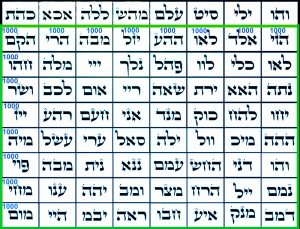Those 72 Triplets are comprised of 216 letters, like the 2160-mile diameter of the Moon, and all this aligns with the diameter of the Sun that is 864,000 mi or (8000 x 216/2) miles.

Moreover, the average distance between the Earth and the Sun is (216/2 x 864,000) miles or (1082 x 8000), and 1082 is 11664, which is (1166411556) or 108 more than the Spherical Time diameter. There is an obvious cosmic or metaphysical relationship between the 72 Triplets and the Sun/Earth/Moon dynamic, and we also see that the Spherical Time bubble seems to be predicated on that same Sun/Earth dynamic, even more so considering their specific surface temperatures (5778 K and 288.9 K) respectively and their temperature ratio 5778/288.9 = 20, as in 203 or 8000.

The Torah for its part has 54 portions, which as we saw earlier are specifically aligned with the 670 paragraphs and the 183 verses. Those 54 portions equal 108/2 and 216/4, while those 80 square roots of the 72 Triplets equal more precisely 1000.054

This all aligns with the complete value of the Ein Sof (אין־סוף), 1660, that is equivalent to (64 x 27.5), the Primal Frequency Sourced from the metaphysical Endless, the Infinite, and the Pure Essence of G-d.

### 10 Generations, One Purpose

The 27 letters of the Alef-bet have a total standard value of 1775, which perfectly matches the 1775 collective years that the 10 generations of Adam lived less than their ideal Binah lifespans of (10 x 1000) = 10000, in other words (100001775) = 8225 years, which is also the number of years that the 10 Forefathers of the 10 generations of Adam lived collectively until the nominal date of the Flood (1656 HC). This is not a causational relationship. It is a painstakingly designed one, like the sum of the 11 sefirot that equals 4225, or 4000 less than 8225, and 2500 more than 1775.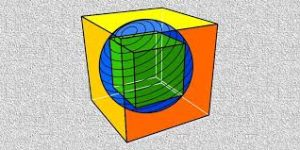While that alignment alone is certainly significant and has important ramifications for the entire Hebrew Calendar that is predicated on those dates, there is a deeper cosmic and metaphysical connection. The ratio of those 8225 years to the Spherical Time radius or Event Horizon of 5778 years, is 1.424, as in the ratio of a sphere inscribed in a cube to the ratio of the sphere that inscribes that cube of volume 1, in other words from cube to cube, 1.424.  It is also the 1424 value of H’Kodesh Kadoshim, The Holy of Holies, the highest spiritual level in the Holy Temple. It is found hidden behind the Gate of 42. And speaking of cubes within cubes, 14.24 is also the cube root of the midpoint in the Spherical Time radius (2889), the midpoint in King David’s 70-year life (2889 HC).

As incredible as this may seem, the difference between the two cube roots of 5778 and 2889 is (17.9442714.24238) = 3.701…, …, in other words, the exact 3701 value of the 42-Letter Name.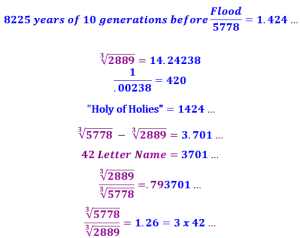Moreover, while the ratio of the cube root of the Spherical Time radius of 5778 to the cube root (14.24238) of the midpoint in the Spherical Time radius (2889) is (17.94427/14.24238) = 1.26, as in the 126-value of the faces, planes, axes, and diagonals of the normal Magical Essential Cube of 42, the inverse ratio (14.24238/17.94427) = .793701, as in the same exact 3701 value of the 42-Letter Name.

### The Primordial Equation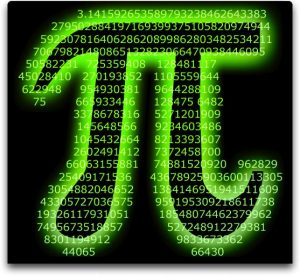A numerical sequence exists within the first 1000 digits of Pi (π): …644622948954

In the middle of it (…644622948954…) is the ratio between the Primal Frequency 27.5 Hz and the Time Coefficient 12 or 27.5/12 = 2.29, but the real key to this sequence is that the sequence breaks down to a set of 4 triplets and the first two, the numeric strings …644… and …622… average out to 633, and thus the 4 triplets give us the exact 3 locations within Pi (π) for the 3 numerical 4-digits strings …5778…., as in the Event Horizon radius.

With the triplets 644 and 622, the locations for the 12 digits in the 3 numerical strings of …5778… form 14 specific digital locations within Pi (π) and thus 14 Triplets in total, which matches the number and ordinal position 14 at the center of the Magic Essential Cube of Creation and of Spherical Time.

These 14 digital location Triplets are 644, 622, 633, 634, 635, 636, 948, 949, 950, 951, 954, 955, 956, and 957, and they sum to 11,424, as in the H’Kodesh Kodeshim, the Holy of Holies (1424).

Yet beyond all reason is the mathematical truth that that sum 11424 is the value of Pi (π) divided by the Primal Frequency, or Pi (π)/27.5 = 3.14159265358/27.5 = .11424

Moreover, that sum 11424 is also equivalent to the 10400 net rows in the Torah plus the 210 word values in it, or (10,400 + 1024) = 11424.

This statistically impossible and metaphysically sparkling sequence …644622948954… is found at the 181st Digit in Pi (π), and 181 is the 42nd Prime Number.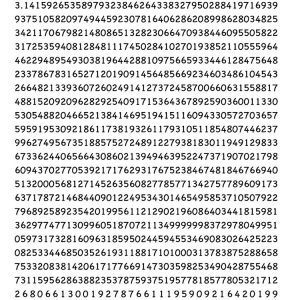Moreover, the difference between the 11,466 square miles of Israel today and the Primordial Equation of the field of Pi (π) divided by the Primal Frequency is (11,46611,424) = 42.

The digit location #948 in these 4 Triplets that begins the sequence …5778185778… and the value of all the digits up to that point is 4240, the value of Moshiach Ben David x 10, the Cosmic Consciousness, while the square root of the value 18 wedged into the sequence is 4.2426, as in Phi (φ)18 = 5778.000.

The digit location #954 in the sequence …644622948954… represents the last 5778 in those first 1000 digits in Pi (π). It also represents the 954 hours that the Israelites waited for Moses until creating the golden calf and short-circuiting themselves and the process.  Their impatience and doubts allowed them to fall 6 hours short of the 40 days Moses and the process was supposed to take. The full 1000 hours as in the 1000 digits is 42 days.

Was it just synchronicity and Cosmic alignment or was it also meant to be foreshadowing?

And it all ties to the Event Horizon 5778, as in the 107th Triangular Number, the square root of 11,466 square miles.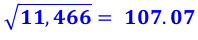Besides 107 being the Sun’s surface temperature (5778 K) and the cumulative sum of the distances of all the planets from the Sun,107 AU, the figure 107 is also found associated with the Sun in the 10.7 cm Radio Flux (sfu) or (2800 MHz), considered one of the most reliable indicators of solar activity. It correlates extremely well with the number of sunspots and with the UltraViolet (UV) and Extreme UltraViolet (EUV) emissions, along with the visible solar irradiance. There is also the uncanny correlation between 107 being the 28th Prime Number.

### A Tale of Two Towers

A cube of volume 27.5 would have an edge or side length of 327.5, making the area of each of its 6 faces (327.5 x 327.5) = 9.110… square units, aligning it with the Torah’s secondary element equation (187 Chapters + 54 Portions + 670 Paragraphs) = 911. Within that 911 sum, we see that the 54 portions of The Torah plus H’Torah (התורה) of numerical value 616, equal (54 + 616) = 670, the number of paragraphs in the Torah, and that the 187 chapters plus the 42-letter Name’s gematria katan, 173, equal (187 + 173) = 360, as in the circle.

And as the puzzle pieces fit together, we see that (911 + 1089) or (911 + 332) = 2000, solving more than one puzzle today if you understand.

The year 2000 CE is the Hebrew year 5760 HC and the log of 5760 is 3.760422, representing the year “0 CE” exactly 2000 years earlier, while the First Holy Temple was destroyed in –422 CE and the Second Holy Temple was destroyed in 70 CE, as in the Hebrew word for seventy (70) that has the gematria value of 422, like the 422 years that the Second Holy Temple stood. The numeric string …2000… is also found in the first 1000 digits in Pi (π) at digit #601, the specific age (601 years) quoted in the Torah for Noach at the time of the Flood and the Great Reset.

There are 48 stories in the Tower of Truth and thus in the Sun Pyramid, and there are 48 verses in the Paragraph of the Flood. They begin with verse #151, as in the value of the Hebrew word Mikve, the ritual cleansing and purification bath, and as in the higher Name of the sphere of Binah, the Name Ehyeh (אלף־הה־יוד־הה).

What is the relationship between the 911 of the “cube of volume 27.5 (1 cubit3)” and 332?

Perhaps we understand and have solved more than we know.

A sphere inscribed in a 1 cubit3 block of stone has a volume of (10 x 332) cubic inches.  This means that a sphere inscribed in the topmost stone of the Tower of Truth and the capstone of the Pyramid of the Sun, would have a volume of (10 x 332) in3. A pyramid with a radiant sphere set into its capstone!

The value 1089 or 332 equals (110011), which is reminiscent of sum of the square roots of the 42 products of the 42 Letters of the 11 sefirot (dimensions) paired with their 42 counterparts in the 42-Letter Name.  They hinted at the Sun’s radius, and they equaled (220022) = 2(110011) = 2(1089) = 2(332).

It is also oddly reminiscent of the two (2) 110-story Twin Towers at One World Trade Center, and of the 40 days and 40 nights of rain during the Flood, seeing as (40 x 27.5) = 1100.

The 11 sefirot (dimensions) of the Tree of Life represent the 32 Paths of Wisdom, so it is also odd that the Tower of Babel incident or second Great Reset discussed in this portion of Noach occurs at the 11th Chapter and the 32nd Paragraph in the Torah, 32 verses before the end of portion—like the 322 word-values in the Torah. The Tower of Babel incident is exactly 112 verses after the Flood Paragraph.  Moreover, the 8 Paragraphs and 112 verses separating the 2 Great Resets or aspects of them are aligned with the 8 first words and/or 33 letters of the Torah that comprise the 11 Triplets of Bereshit.

The first 31 Paragraphs until that moment of the second Great Reset total 267 verses leaving 1267 verses in the rest of the Book of Genesis (Bereshit), aligning them with the hypotenuse of the Tower of Truth and the apothem of the Pyramid of the Sun.  The Twin Towers were replaced by the 8-sided octagon shaped One World Trade Center that is 1776’ tall, as in the year 1776 HC, when the Tower of Babel reset occurred, 120 years after the Flood reset in 1656 HC, as in the 8th Triangular Number, 120.

Another mysterious piece of this puzzle is hidden in the 72 Triplets. The square roots of each of the 9 column totals add up to 267.7… and because the square roots of its 8 rows and 72 individual Triplets equal 1000.054, the total for the 90 square roots is 1267.7, perfectly matching the verses split (2671267) in Genesis at the Tower of Babel event.  We can philosophically and metaphysically extrapolate that since the 72 Names comes from the Splitting of the Red Sea event and the 3 verses of 72 that the two events are bound together. Moreover, like the sum of the initials of the 72 Triplets, 2672 there are the 672 years between these important events in 1776 HC and 2448 HC. They are split into 224 and 448 years by the year 2000 HC, exactly a 33.3% and 66.6% split. Also, the 9th plague, darkness, Choshech (חשך), has a numerical value of 328, the complement to the 672 years in that (328 + 672) = 1000 like the sum of the square roots of the 9 rows and 72 Triplets in the 72 Names that total 280.0 + 720.0) = 1000.0.

The value 1776 is also found as a numerical string …1776… within Pi, 3.14…171776 at digit #888, exactly one half of 1776, and 112 digits before 1000. It is also the exact value of the Torah’s 4th verse: “And God saw the light, that it was good; and God divided the light from the darkness.” The word for “good, tov (טוֹב) has the value of 17, as in the “17” that precedes …1776…. in Pi (π).

### וַיַּרְא אֱלֹהִים אֶת-הָאוֹר, כִּי-טוֹב; וַיַּבְדֵּל אֱלֹהִים, בֵּין הָאוֹר וּבֵין הַחֹשֶׁךְ.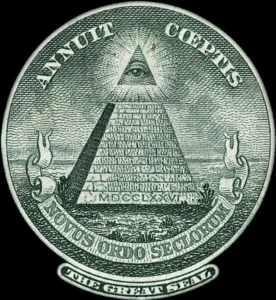Then again, whether you see a connection to the back of the Great Seal on the US \$1 bill having a pyramid of 72 bricks engraved with 1776 in 3 triplets of Roman numerals (MDC, CLX, XVI) whose backs total 666 or not above the words New World Order is a personal preference.

Nevertheless, the first verse of the 3 consecutive verses of 72 letters has a numerical value of 2672 and the final one of 3428, leaving a difference (34282672) between them of 756, as in the 756 ft base of the Pyramid of the Sun and the 6 faces of the Essential Magic Cube of Creation. Their total (3428 + 2672) = 6100 or (100 x 61), the value of the middle or 37th Triplet (אני) of the 72 Triplets, and it is (10 x 610), the 27th Triplet (ירת), which is the value of the 3rd tier of the 42-Letters of the YHVH (יהוה) and the 16th Fibonacci Number. By the way, that Seal with the truncated pyramid was already in circulation on the \$50 note by 1778.

### The Scroll of Esther

It is well known that the Megillat Esther is all about concealment and that there are hundreds of instances in which the Names of G-d are concealed within it.  In the 9th chapter of that Scroll of Esther there is a well-known series of two-word paragraphs listing the 10 sons of Haman that were hanged. This series that stretches from verse 710 must be read in one breath. Its 11 paragraphs stretch from the 17th to the 27th paragraph and end with the words “the 10 sons of Haman (עשרת־בני־המן)” of numerical of 1777 or (1776 + 1).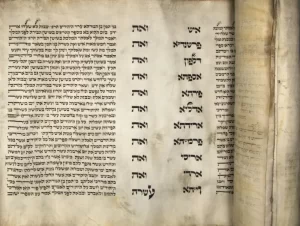The full breakdown of the paragraph schematic is:

26 verses in the first 5 paragraphs (15);

26 verses in the next 3 paragraphs (68);

42 verses in the first 6 paragraphs (16);

91 verses in the first 10 paragraphs (110);

73 verses in the next 6 paragraphs (712);

26 verses in the next 14 paragraphs (1326);

26 verses in the last 3 paragraphs (2729);

28 verses in the last 12 paragraphs (1829)

Nevertheless, paragraphs 17 27 comprise two special columns. The final words, or the second column, is comprised of 11 of the 22 words, split down the middle like the 22 letters of the Alef-bet into odd-even. It totals 5040, as in the Earth and Moon radii, 5040 miles, and as in 7! factorial.

As for the first 11 names/words in the first column, they all begin or end, or begin and end, with the letter Alef (א) except the 3rd one of numerical value 170. They contain 13 Alefs (א) in total, all as one, echad, which is like the 130 value for the 11 initials of the second column of 11 words. Altogether there are 91 letters in these 11 rows/paragraphs, as in the Path of One and in the concealed unified Name of the Creator, and the 91 verses in the Scroll’s first 10 paragraphs.

The 11 initials of the first column total 256, or 28 as in “Aaron (אהרן),” whose name also beings with Alefs (א) and who lost his sons, and who is the only one in the torah titled Moshiach.

While the katan value for all 91 letters plus the kolel is (309 + 91) = 400, the 57 letters in the 11 names/words of the first column total 5358, as in Moshiach (358) and as in 420 years prior to 5778. Meanwhile the final letter of each of the 11 rows in the 2 x 11 matrix is Tav (ת) of numerical value 400. What makes this even more extraordinary is that the ordinal value of those 11 names/words is 633, which matches the sum of their initials and final letters (256 + 377) = 633, and while 377 is the 15th Fibonacci Number, it is at digit #633 in Pi (π), where we first encounter the numeric string …5778…. Moreover, their ordinal sofit value is 644, as in the digital sequence …644622948954… found at the 181st Digit in Pi (π) that indicates where to find all 3 sequences of …5778…. Then there is the milui or spelled-out value of the 11 names/words in the first column, or 11,567, which is 11 more than 11,556, the diameter of Spherical Time that is twice 5778.

We are told that after the arrival of Moshiach Consciousness, the only books necessary will be the Torah and the Megillat Esther and the total gematria milui for all 22 words or 91 letters is 18,363, as in H’Moshiach (363) and 18 whose square root is 4.2426, Moshiach Ben David, the Cosmic Consciousness.

Whether there is meaning in this or not, the total ordinal value for the 91 letters is 369 like the constant (369) of the 9 x 9 normal magic square, and like the constant (34) of the 4 x 4 normal magic square that aligns with the 34 letters in the second column or set of 11 words.

Furthermore, those 11 final letters of the 2nd column total 4400 or (4 x 40 x 27.5), and the value or year 1776 is equal to (4 x 444), as in the value of the Torah phrase “from generation to generation (לדר־ודר),” which as Rav Ashlag pointed out to me aligns with the equation 444/22 = 20.18.

The number and year (1776) is also equal to (2 x 666 + .666 x 666) in the same way that the Physical Time radius 2018 CE, the Western analogue to 5778 HC, equals (3 x 666 + 3 x 6.66) = 3(666 + 6.66).

### The Circle of 42

While the 40th Prime Number is 173, as in the katan value of the 42-Letter Name, the 248 dimensions of the E8 Lattice, the Torah, etc. when divided by 137, the 33rd Prime Number and/or the Fine Structure Constant is 248/137 = 181.0 and 181 is the 42nd Prime Number, 8 more than 173. It is also the sum (181) of the first 42 digits in the set of all Prime Numbers, and it is notably 112φ, which is not only representative of the 112 Triplets, but the sum of the 8 corners of the Essential Magical Cube of 42.

The Torah connects 181 with 42 and with 72 through the word value of 16, which are like the 2 masculine letters YV (יו) in the YHVH (יהוה). The word value 16 is found 72 times in the Torah, and the cube root of 16 times 72 is 181.4286… Moreover, the spelled out letters Yud Vav (יו) have the numerical value 42.  What takes this next level is that the 1600th YHVH (יהוה) in the Torah is found at Shoftim 18:1 and Shoftim, the 48th Portion in the Torah begins with verse 16:18 as in Phi (φ), and there are 16 empty rows between the Books in the Torah.Meanwhile, the sum of the digits through 181 (the 42nd Prime Number) is 378, the 27th Triangular Number associated with the Essential Cube of Creation and the Alef-bet. Through each of the 26 outer points of the Cube there are set vectors through the center, 13 if we disregard their direction. The 3 axis vectors can only go through the center position (14) while any other number can begin multiple surface vectors plus one through the center. Those 3 axis vectors begin at points 1, 3, and 7 as in 137 and as in 173.

And the cumulative 42 cumulative Prime Numbers, in other words the 42nd Tetra Prime, equal 45,903, as in the number 903, the 42nd Triangular Number.

Meanwhile, the Tower of Truth and Pyramid of the Sun are 20 stages or tiers high, and the 20th Tetra Prime is 4200.

What brings this full circle is that this sum of the 42 cumulative Prime numbers, 45,903, equates to 333.84 cycles of 137.5o, and to 3338.4/2 cycles of 27.5o, thus aligning the Singularity of 42 with the year 3338 HC when the First Holy Temple was destroyed, and with (5778 x .5778), which equals 3338.5284, or 422 years before the pivotal year (3760 HC, 0 CE).

This date of the 5th month of 3338 HC is also given by the surface area of the Spherical Time Sphere at the year 2889 HC, the midpoint in King David’s Life and in the Event Horizon. The formula for the surface area of a sphere is 4πr2 and thus the 4(2889)2 radial component in it = 33385284. Part 3 will follow shortly…